# ML Aggarwal Solutions for Class 10 Maths Chapter 14: Locus

ML Aggarwal Solutions for Class 10 Maths Chapter 14 Locus is the prime pick for students looking for the best books. Students are highly recommended to refer to the solutions and solve problems as it covers the latest syllabus of ICSE board. To score well in the annual exam, ML Aggarwal Solutions for Class 10 Maths Chapter 14 Locus PDF can be downloaded from the links, which are available here.

Chapter 14 explains the concept of locus and various terms which are important from the exam perspective. The solutions designed help students prepare for the board exams and competitive exams at various levels. PDF comprises ML Aggarwal Solutions that will build a strong base of topics, which are covered in this chapter.

## PDF Solution for ML Aggarwal Class 10 Maths Chapter 14 :-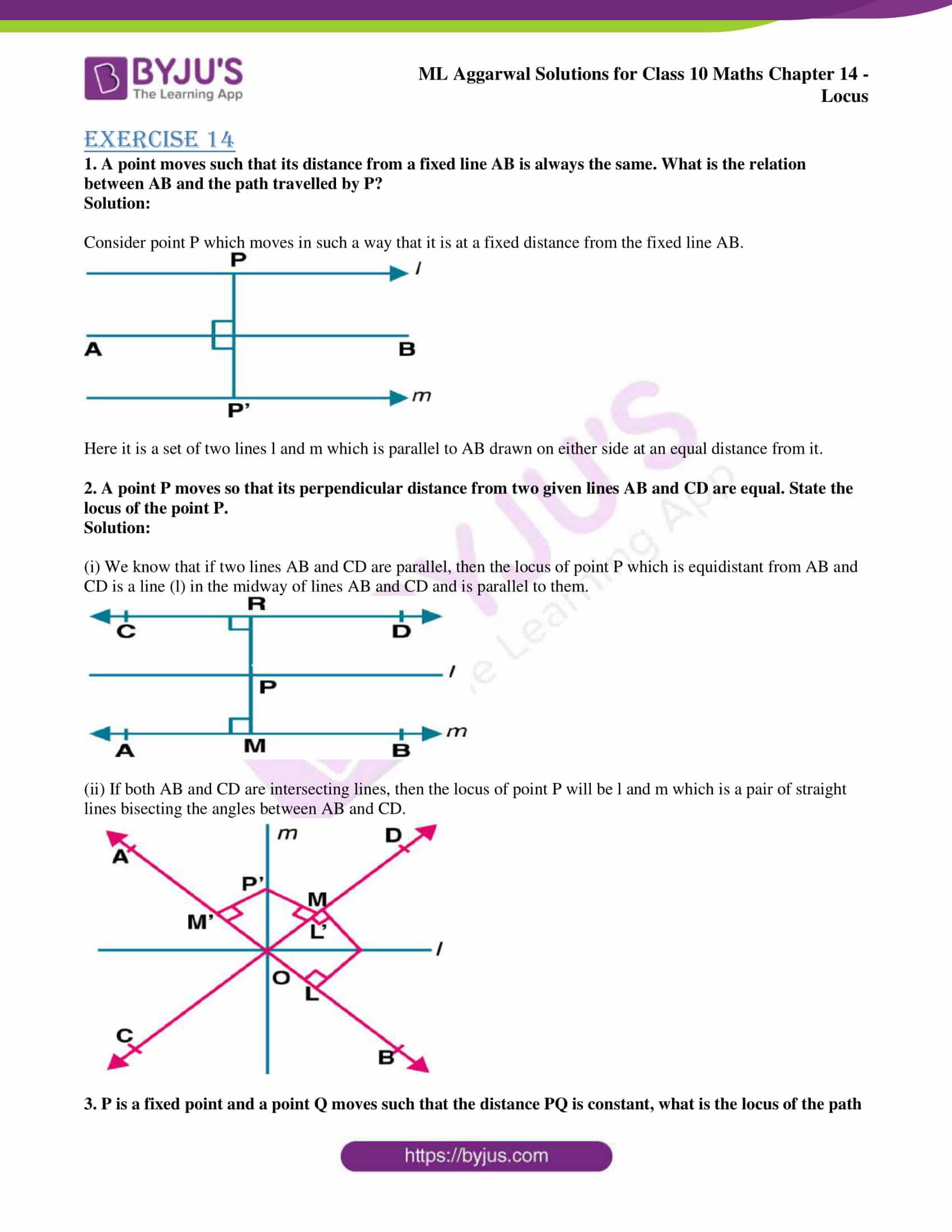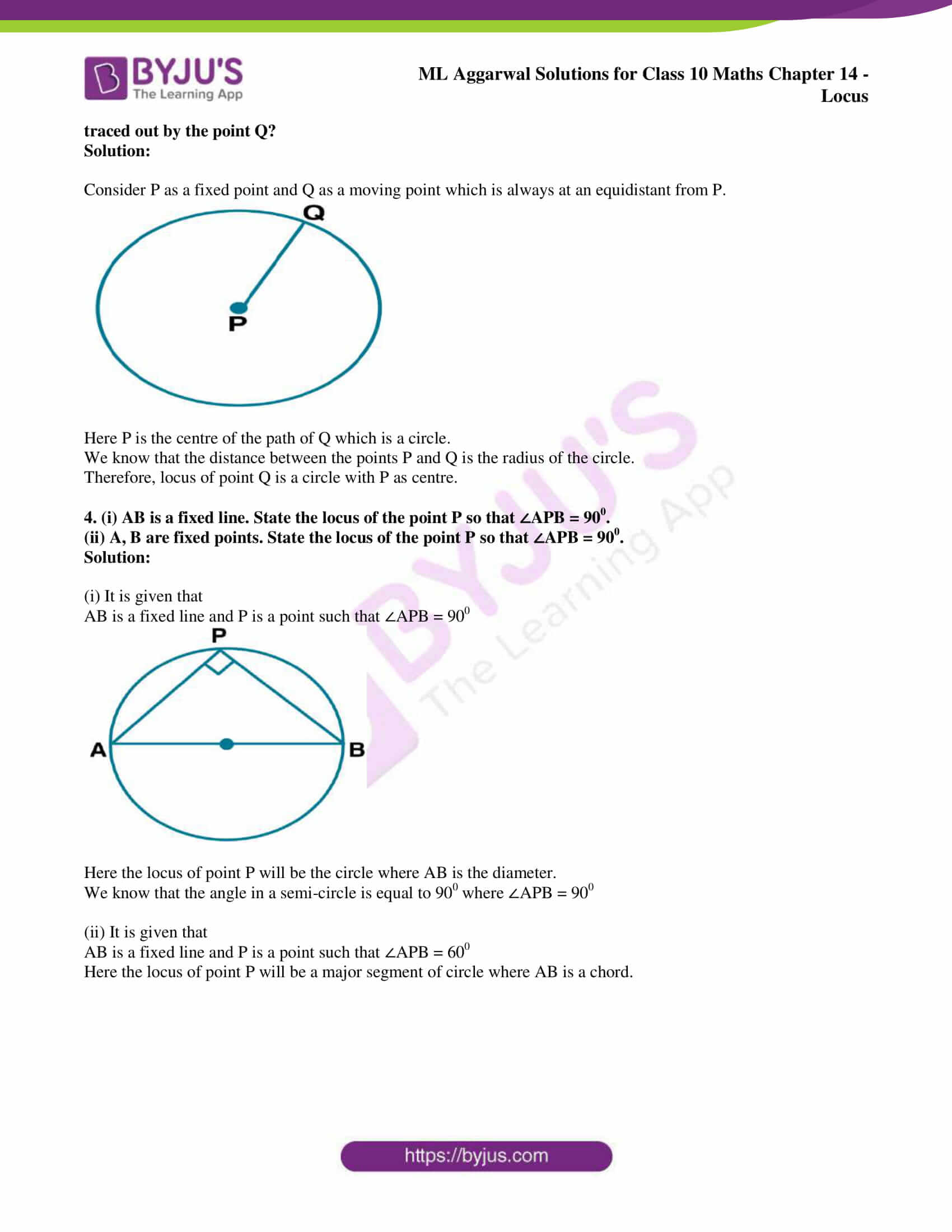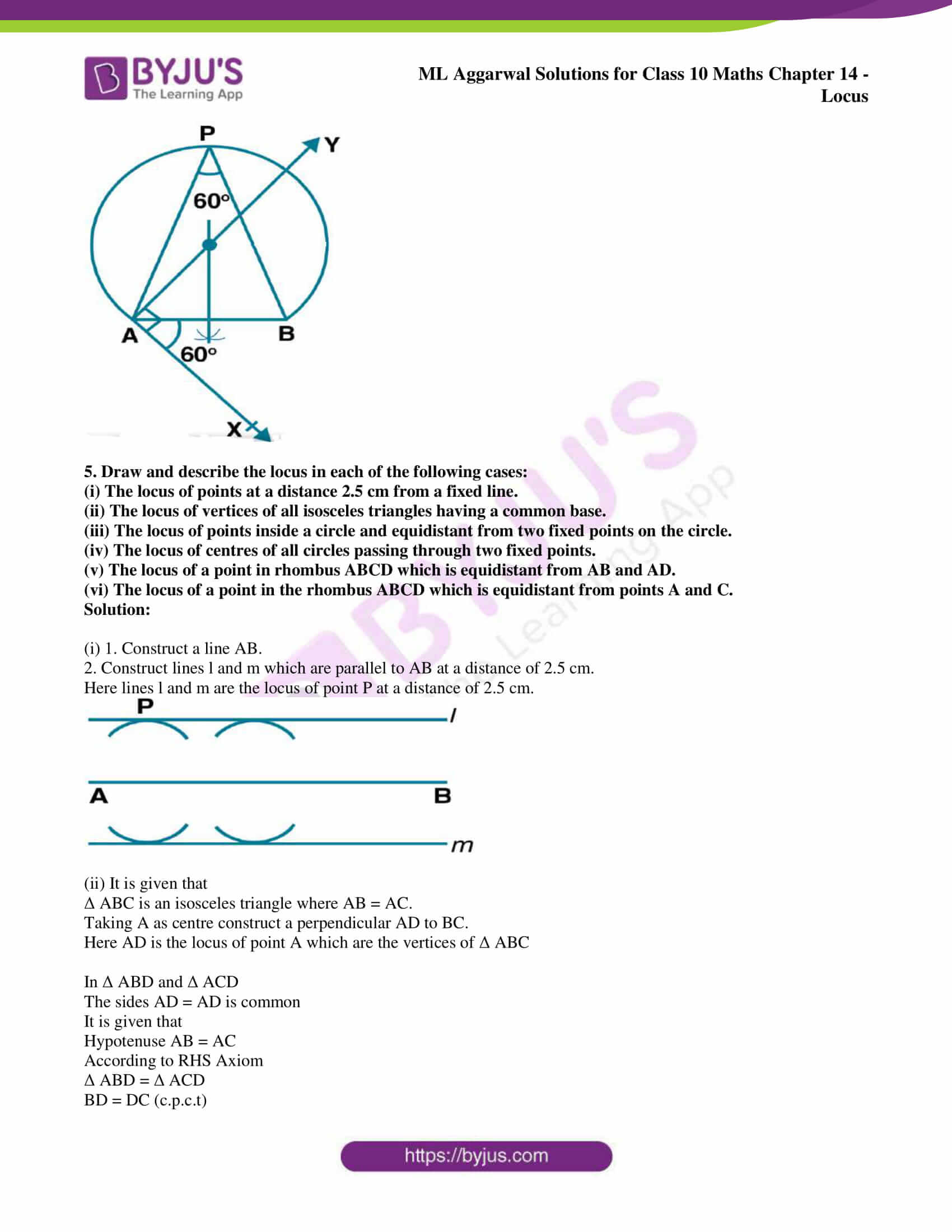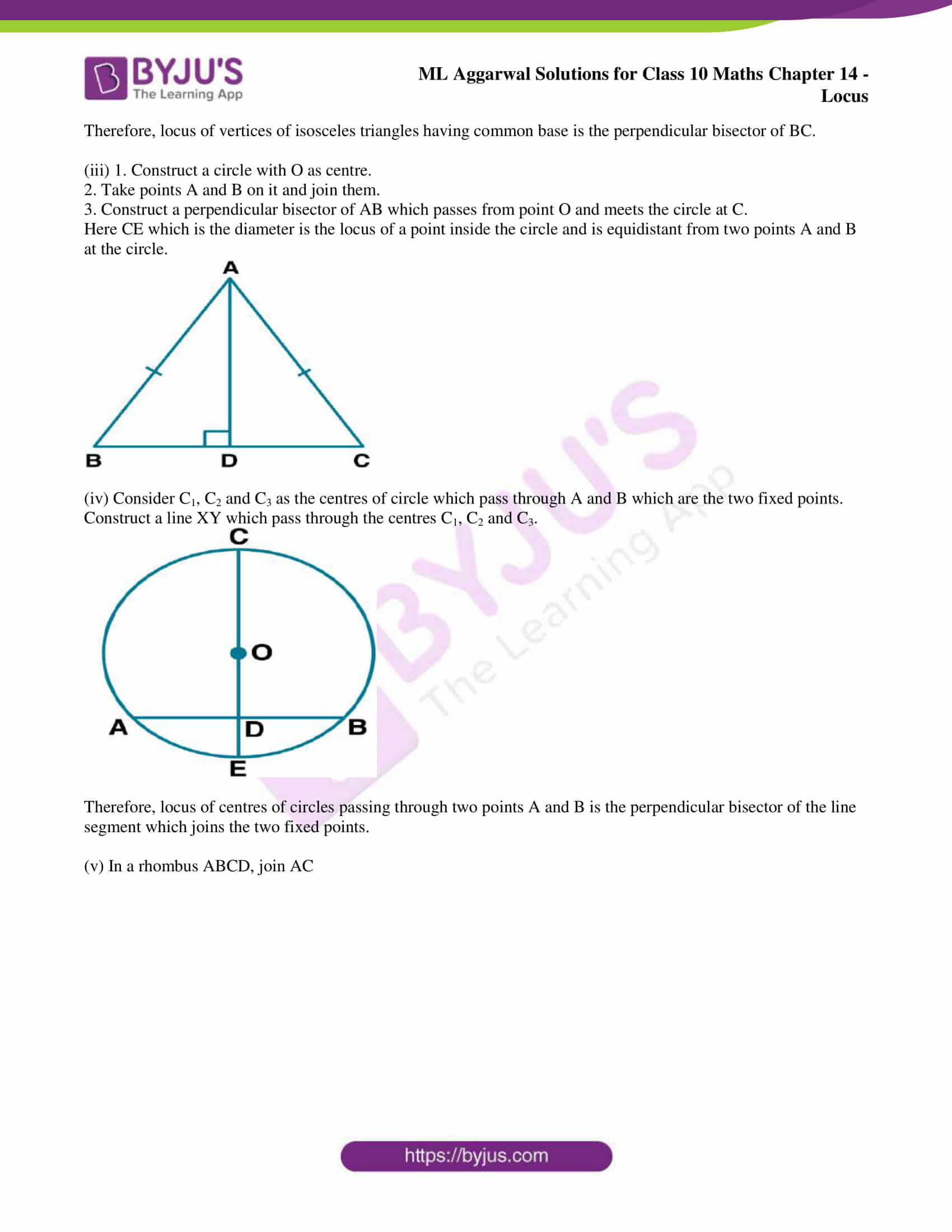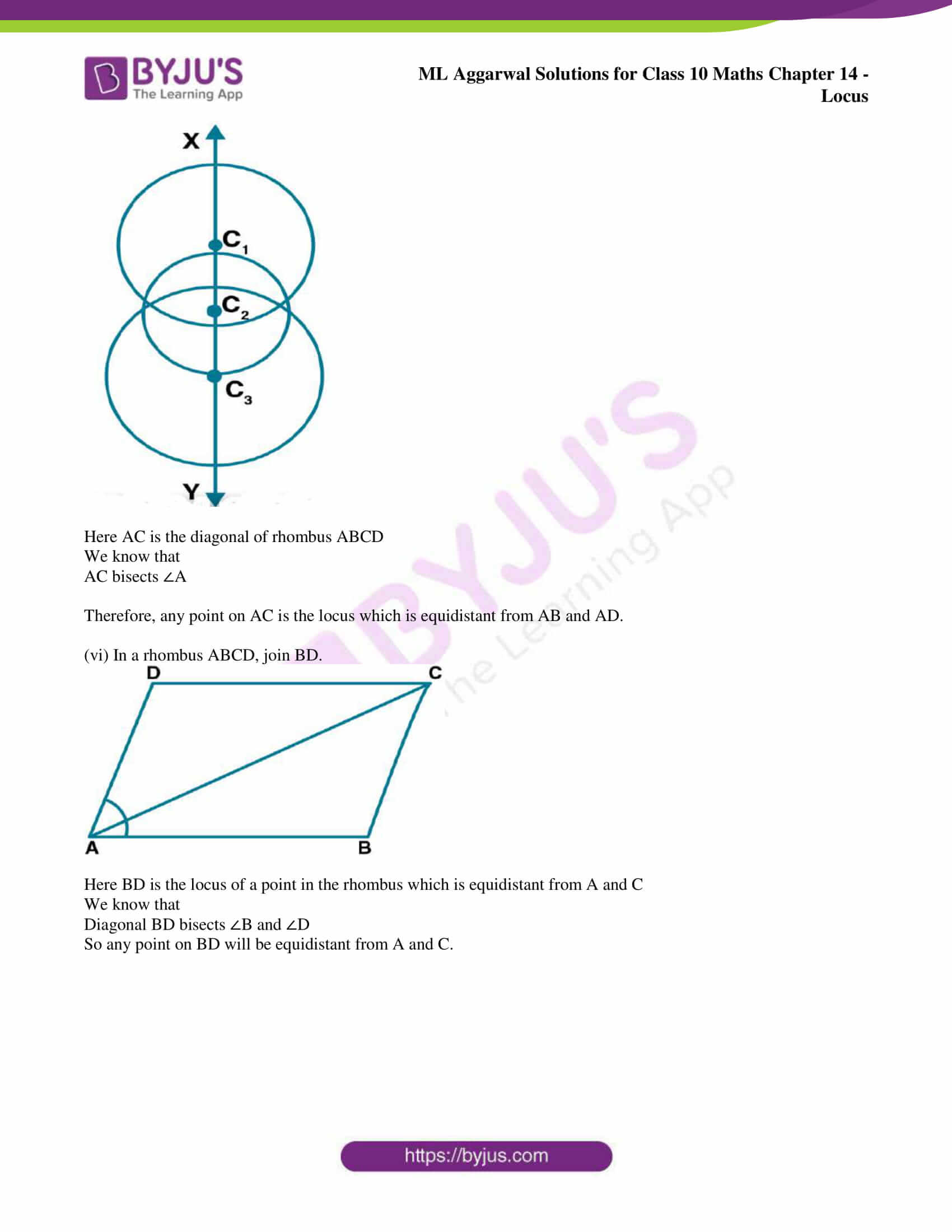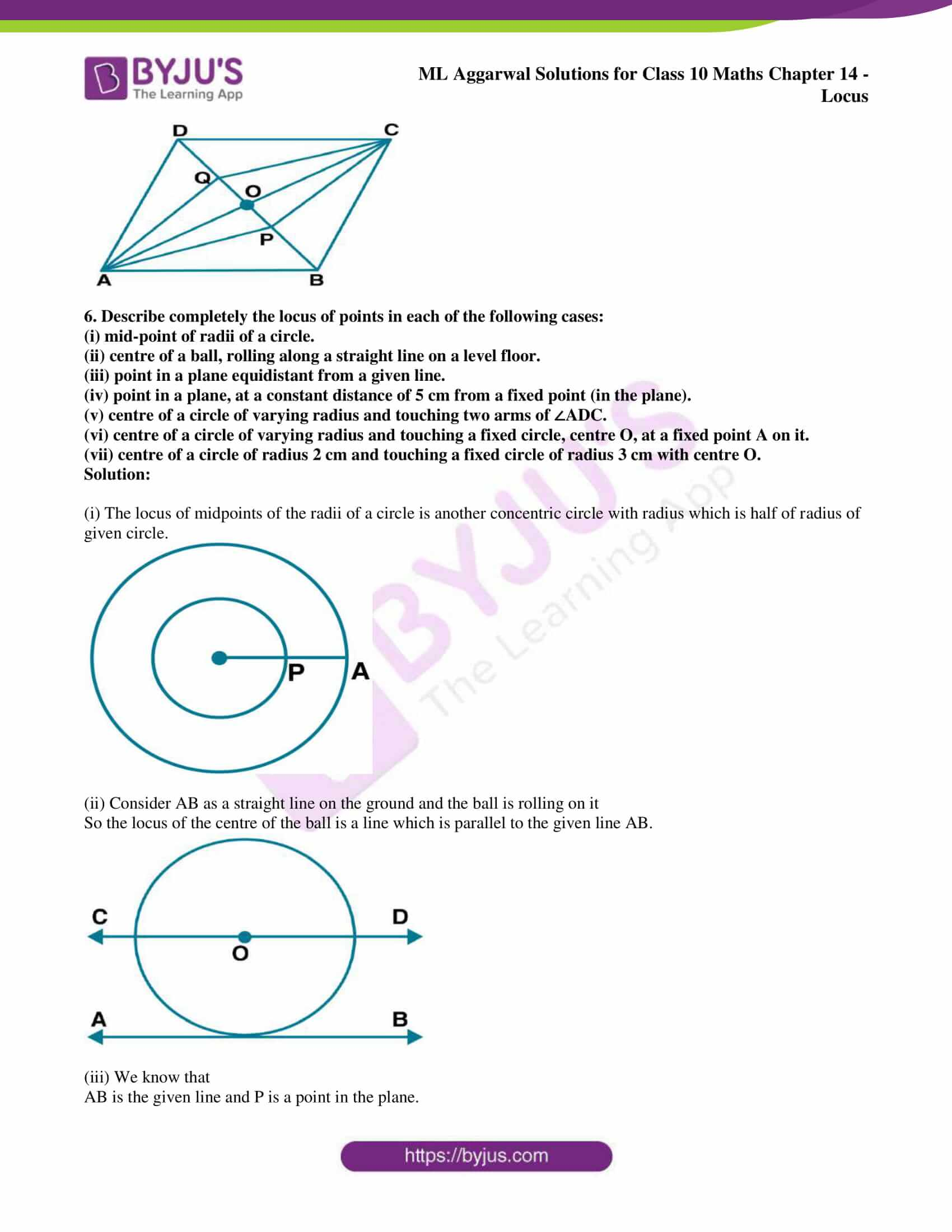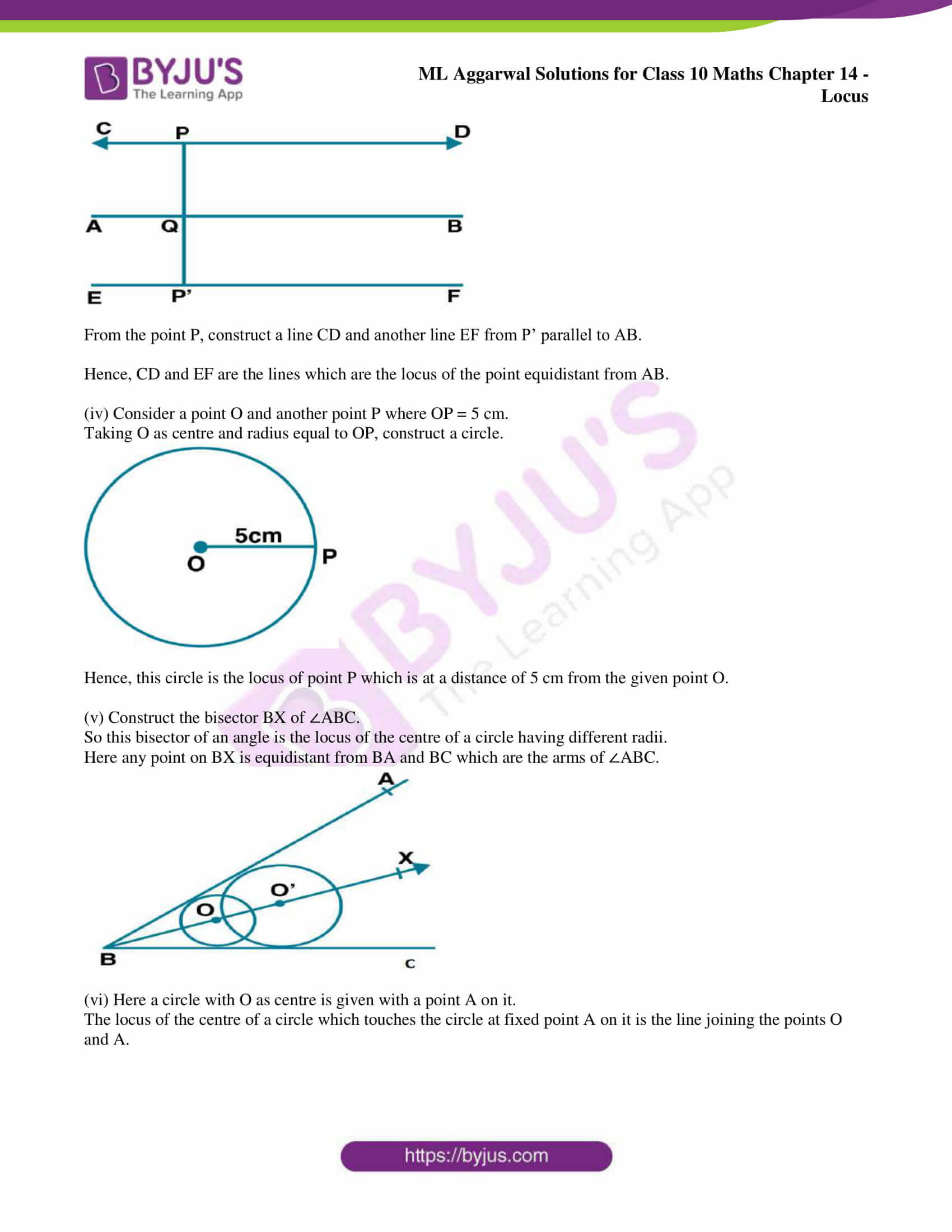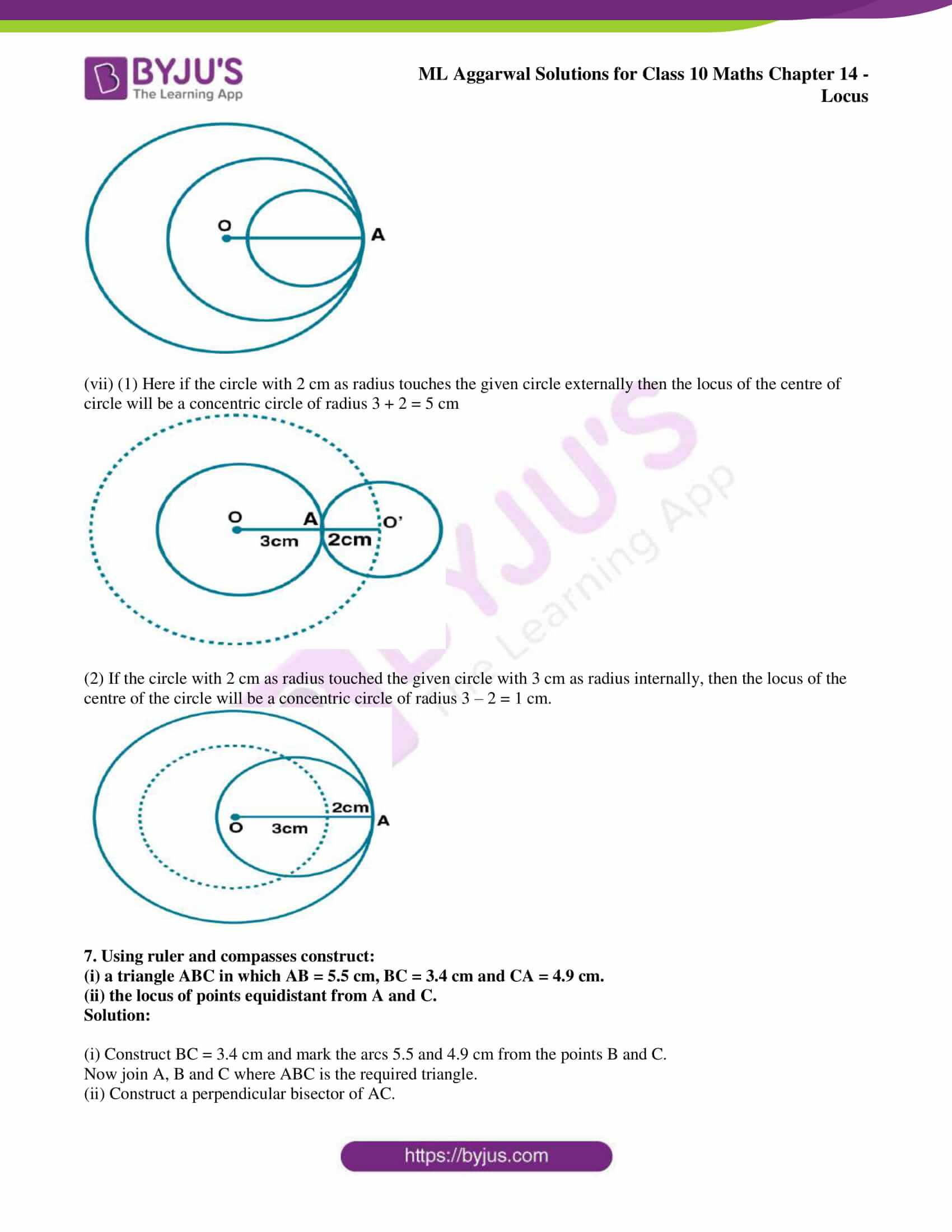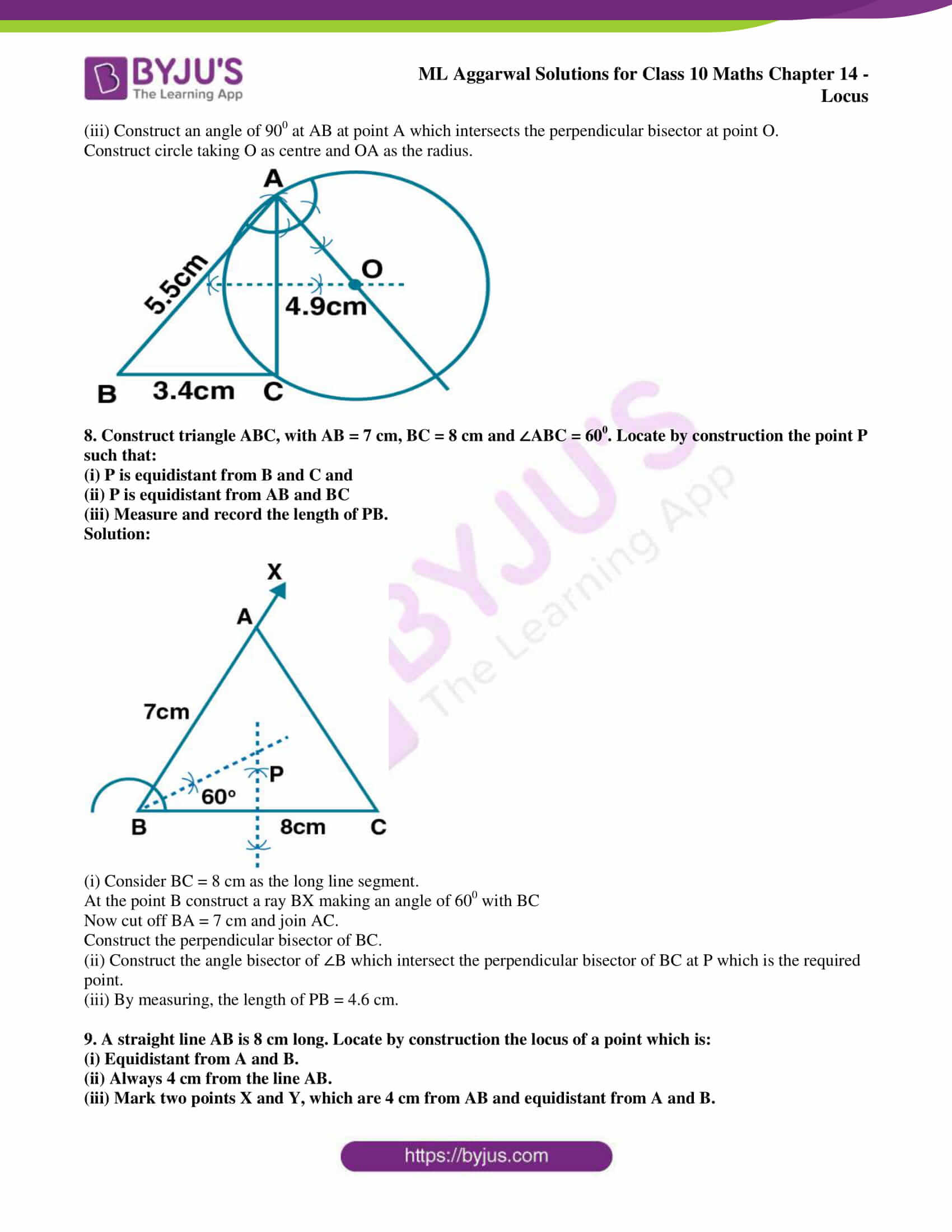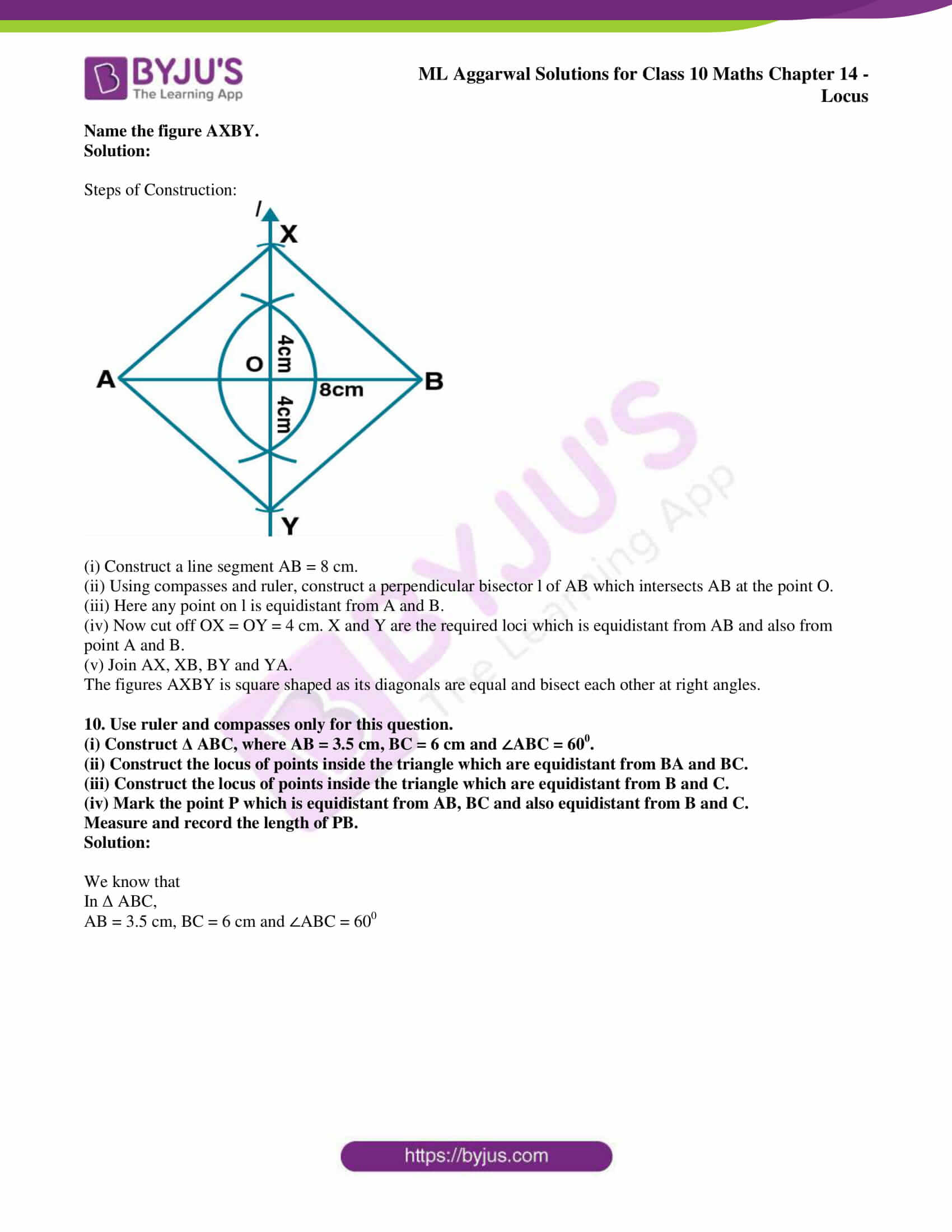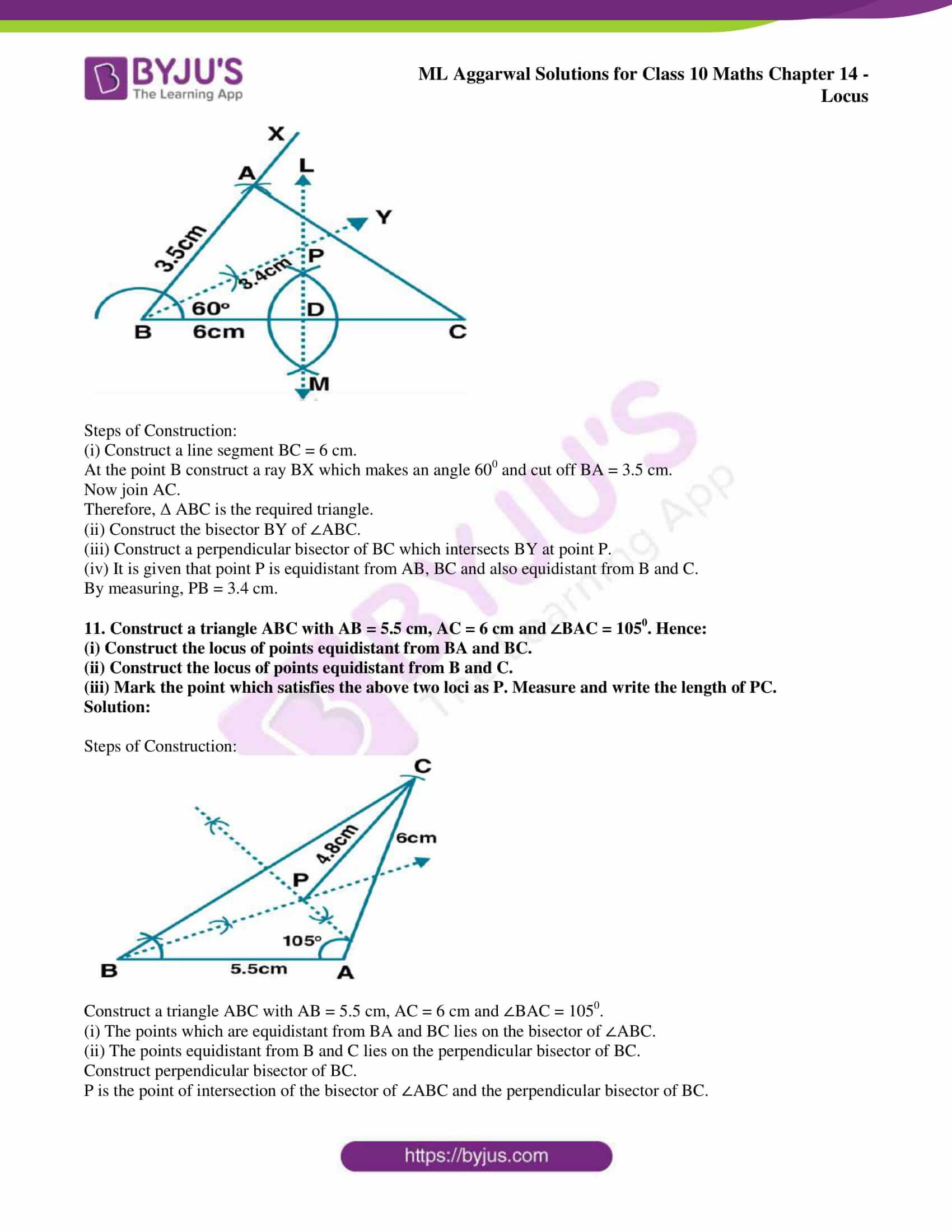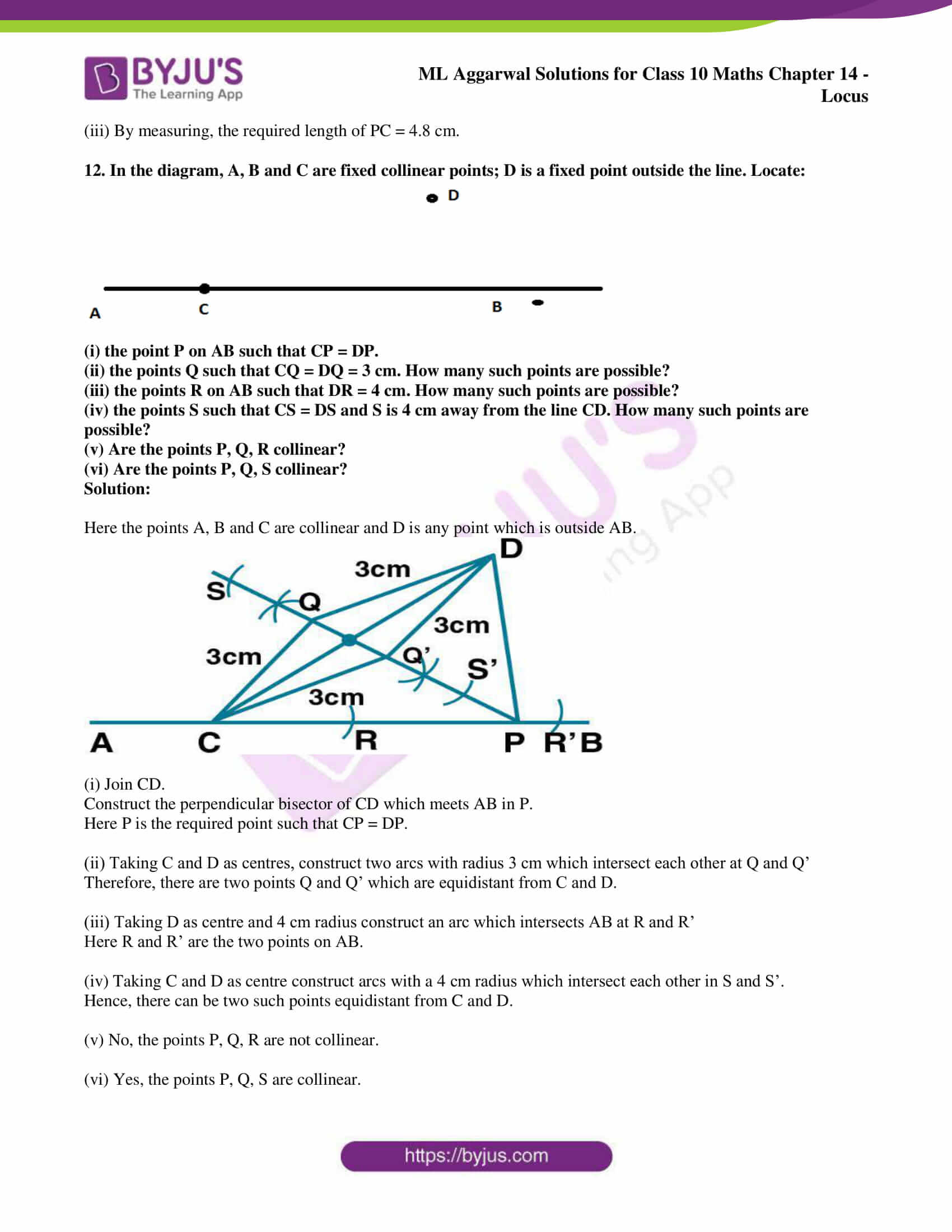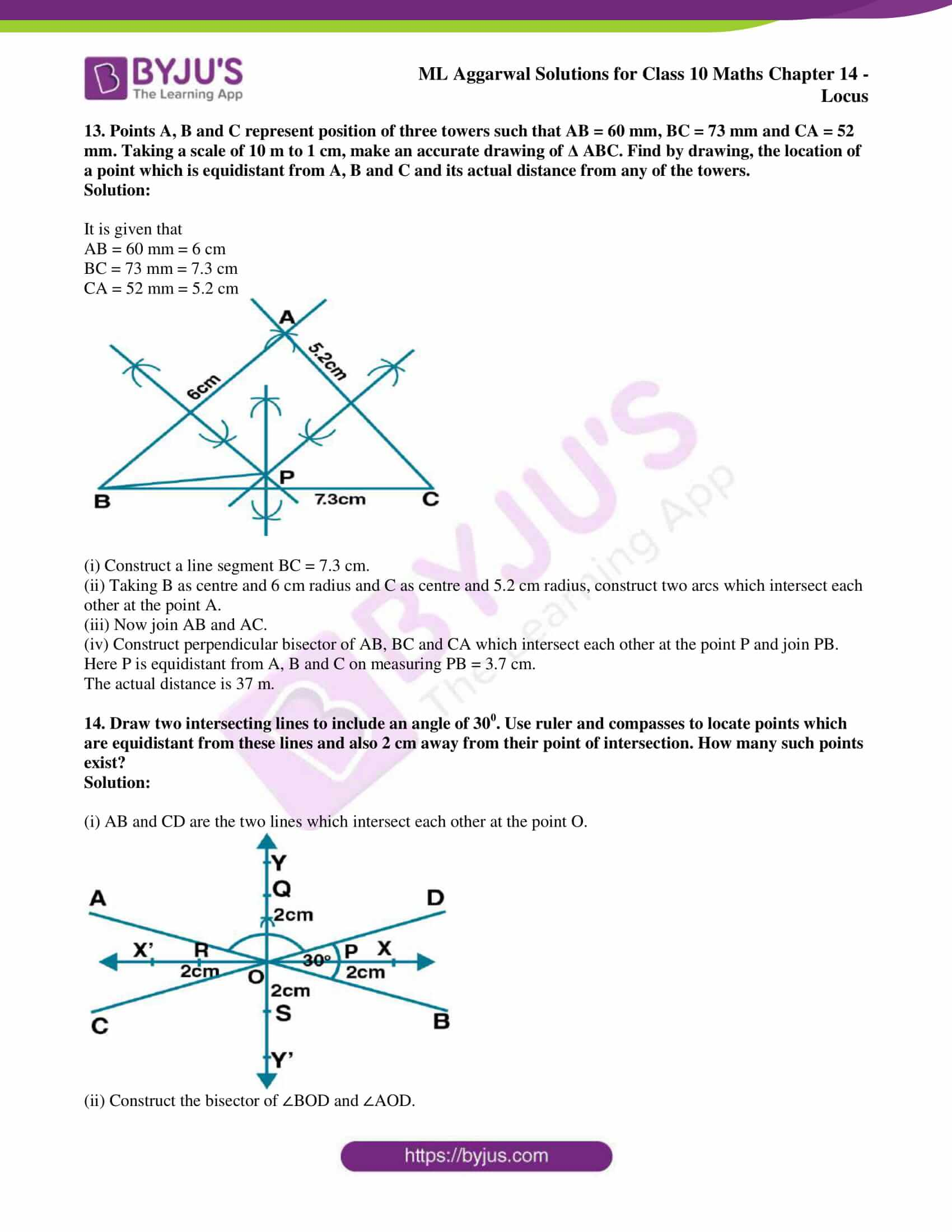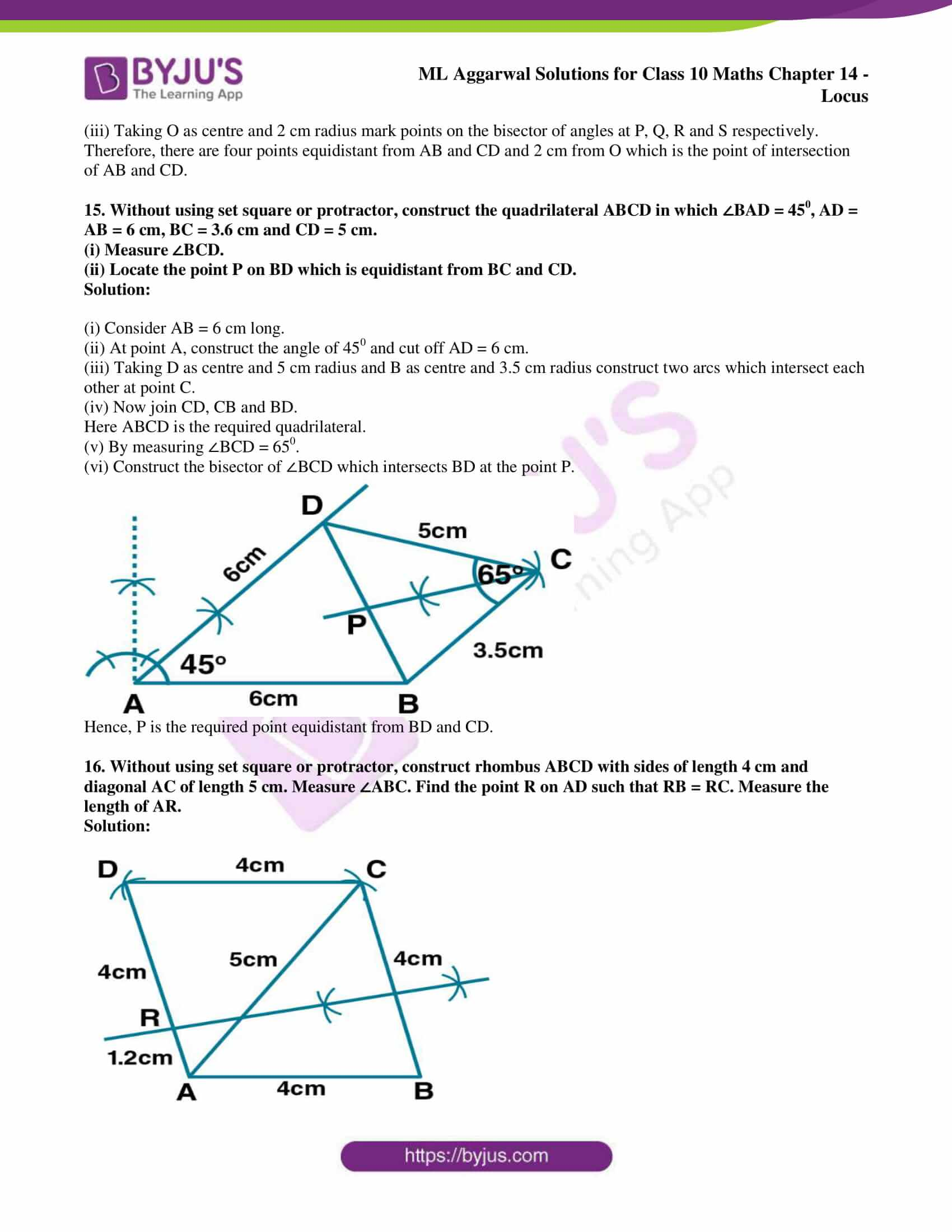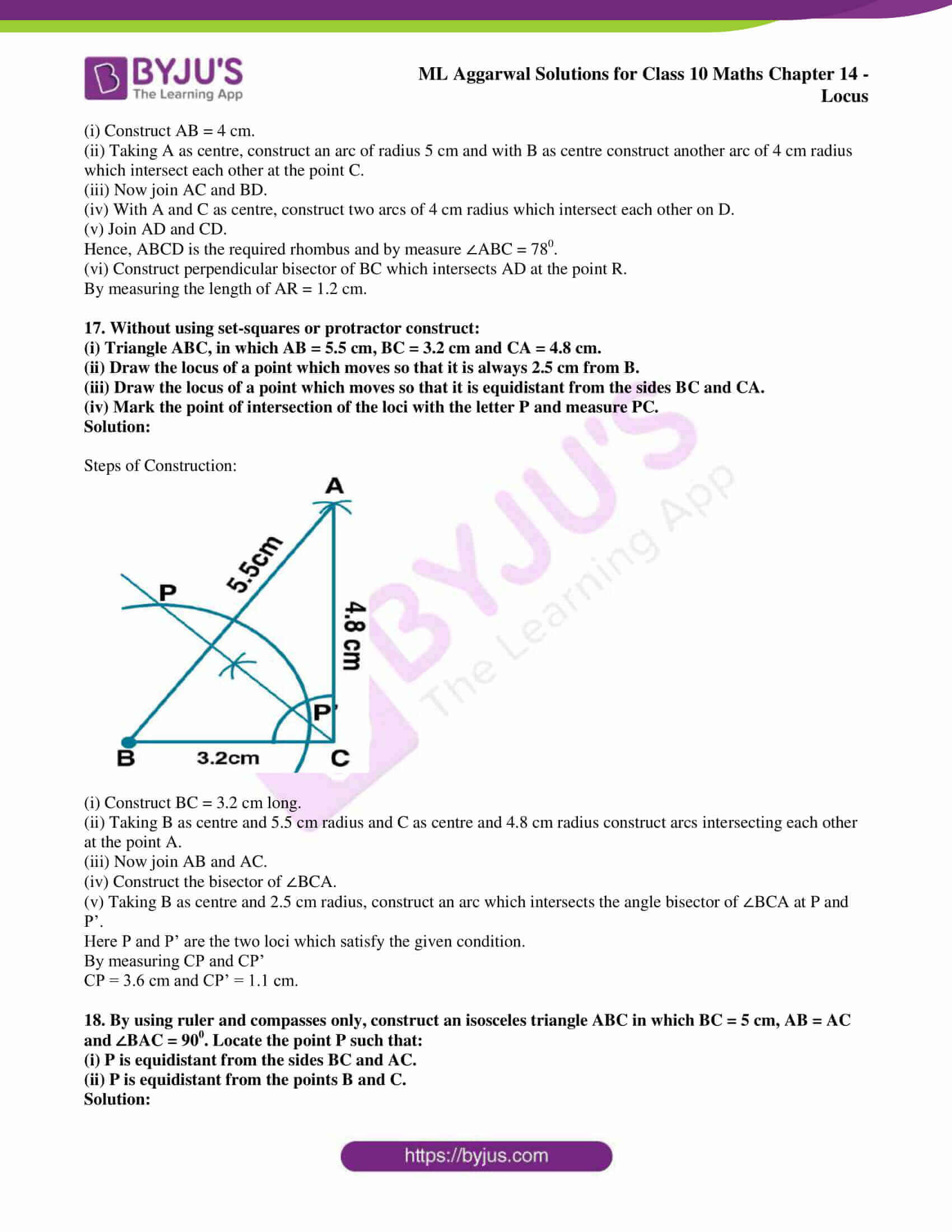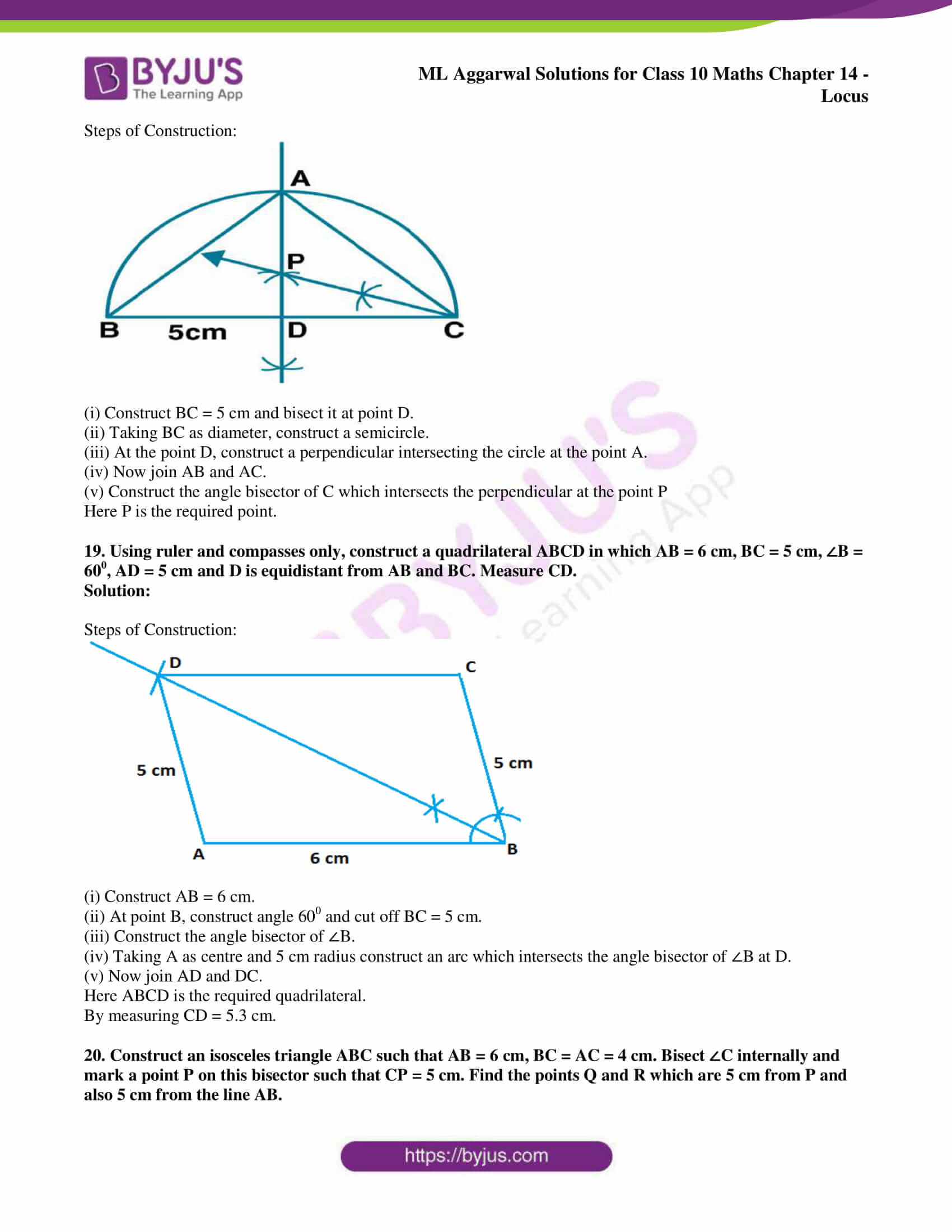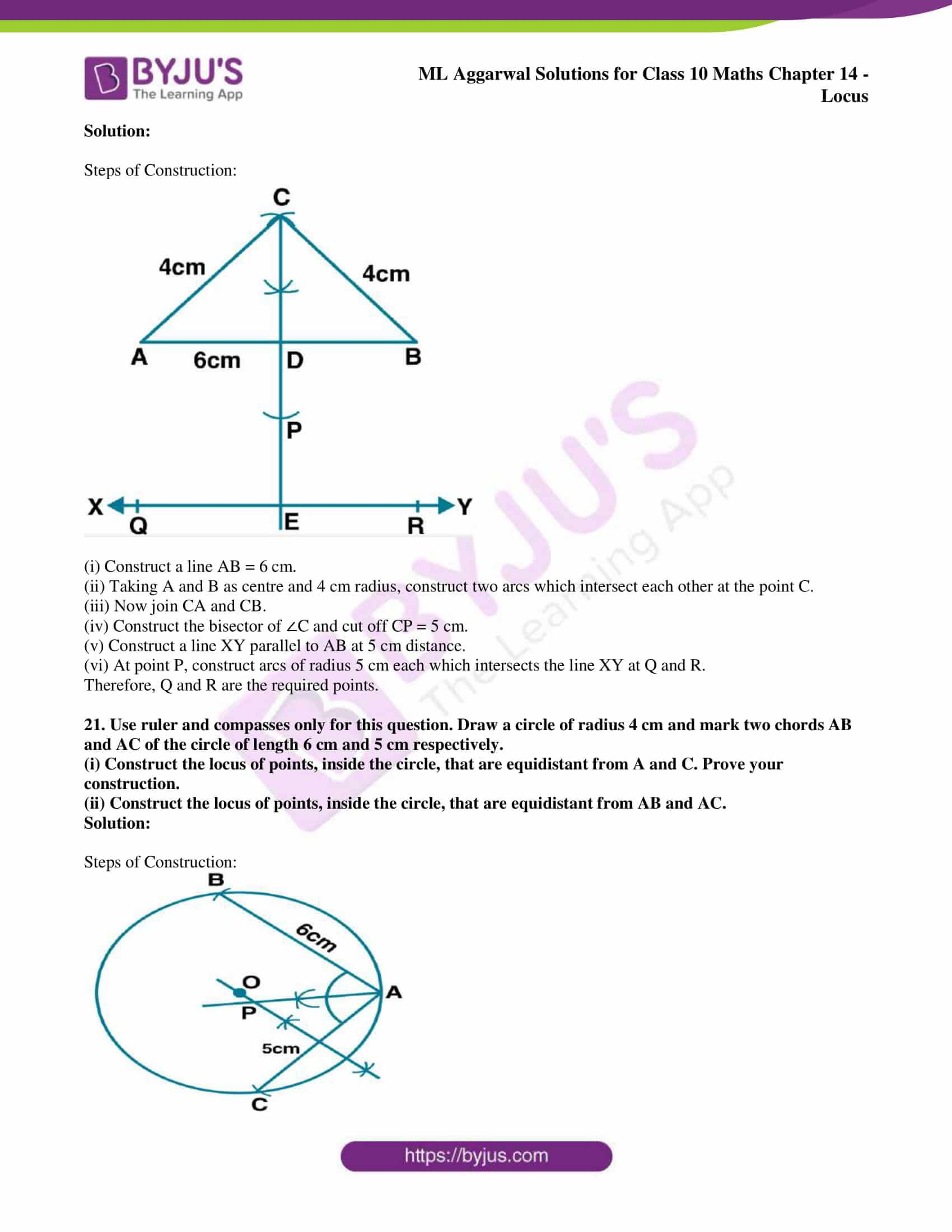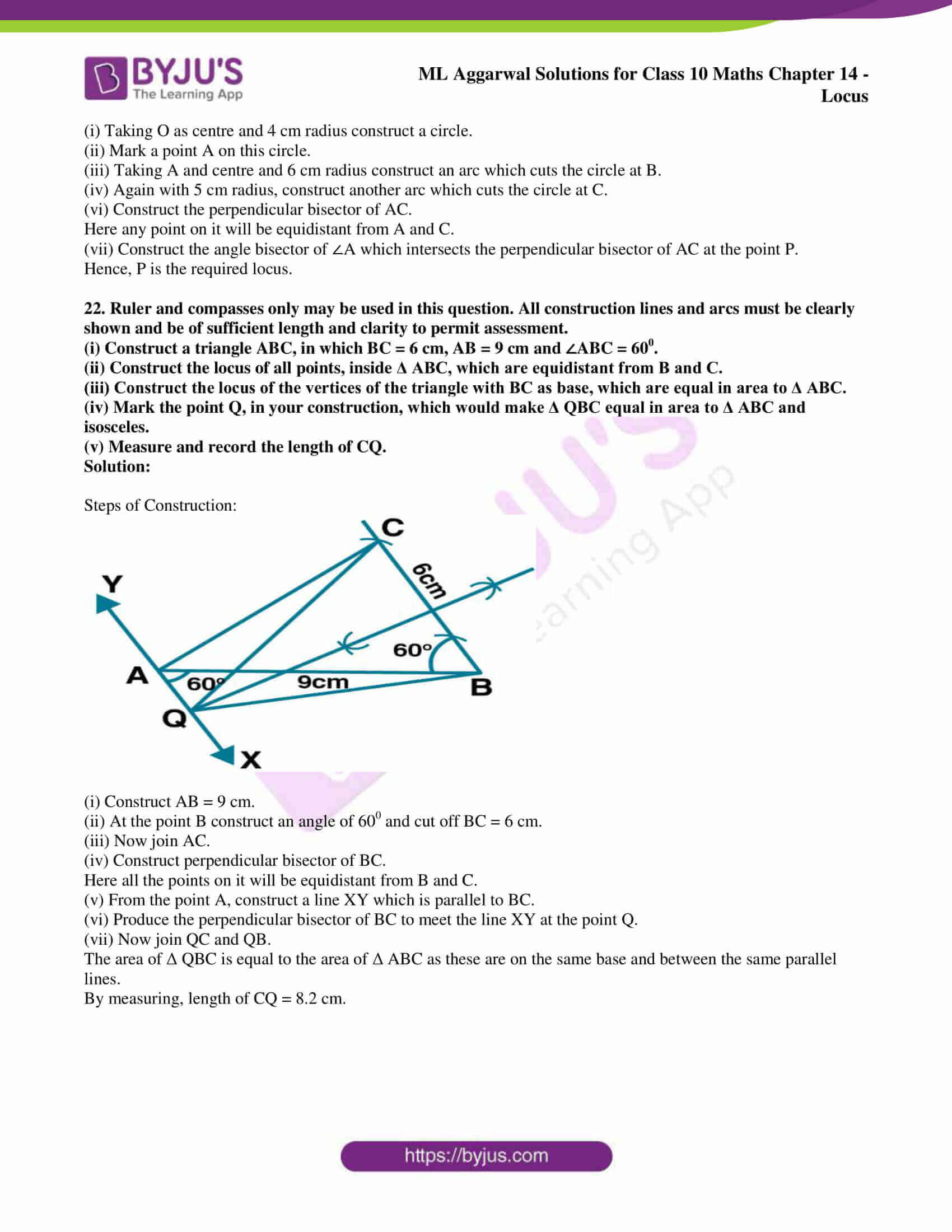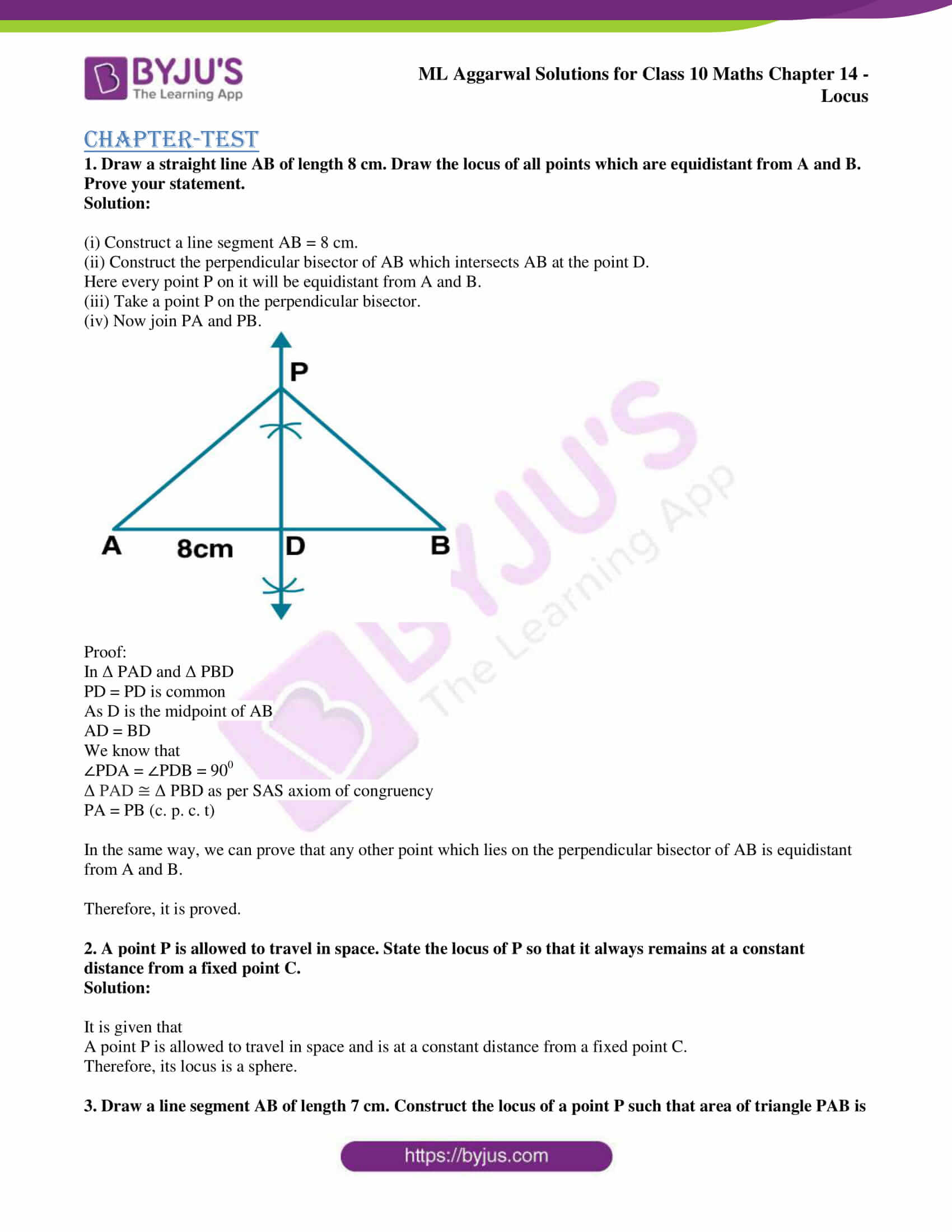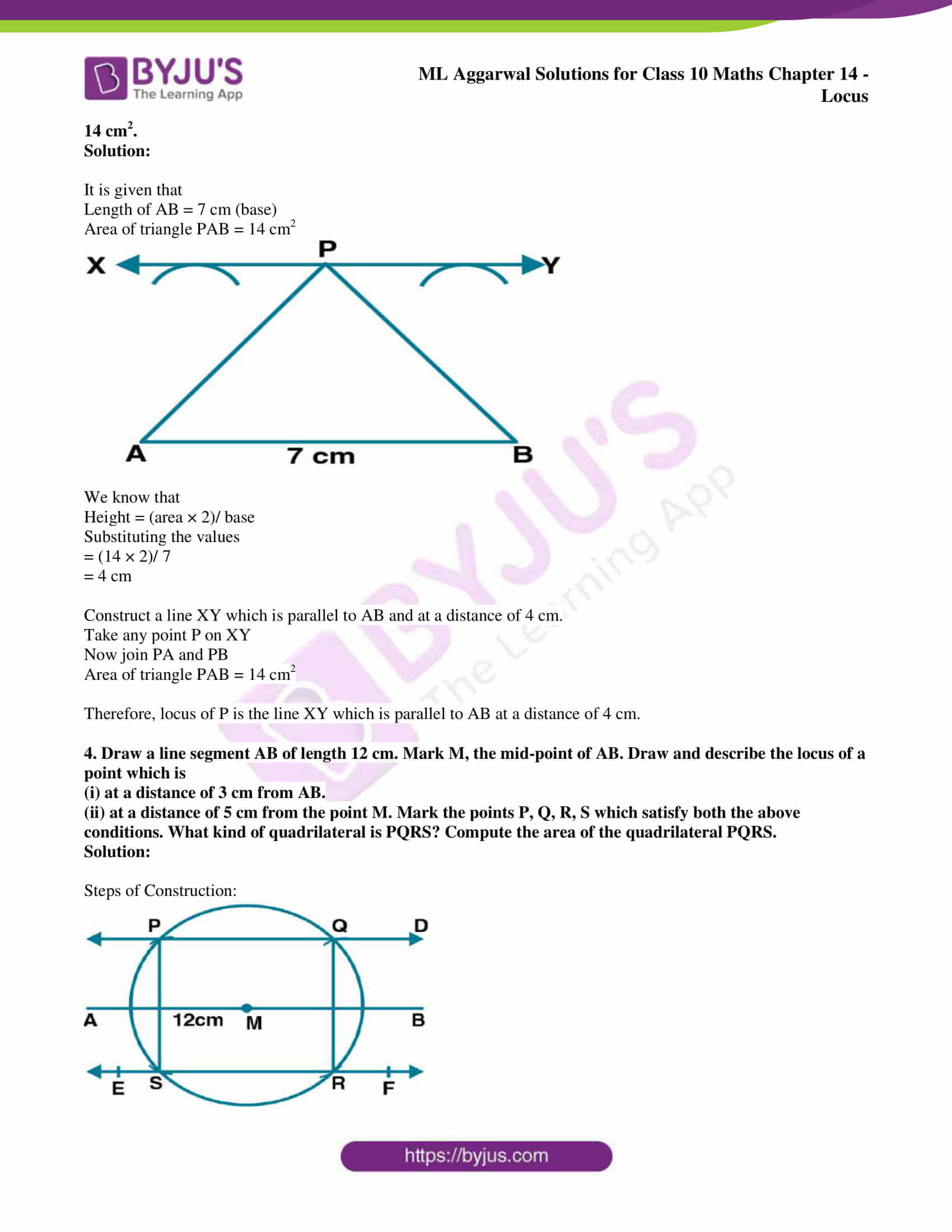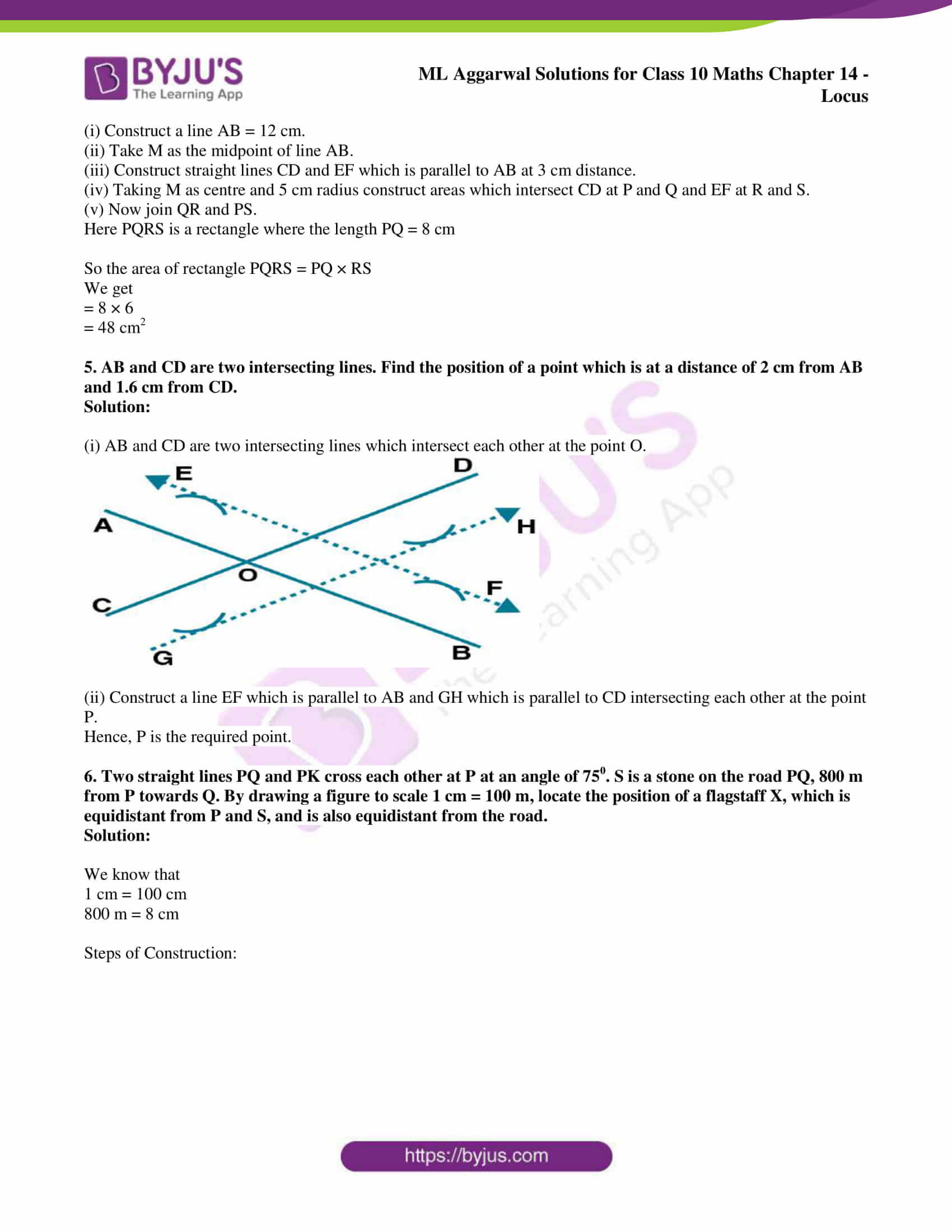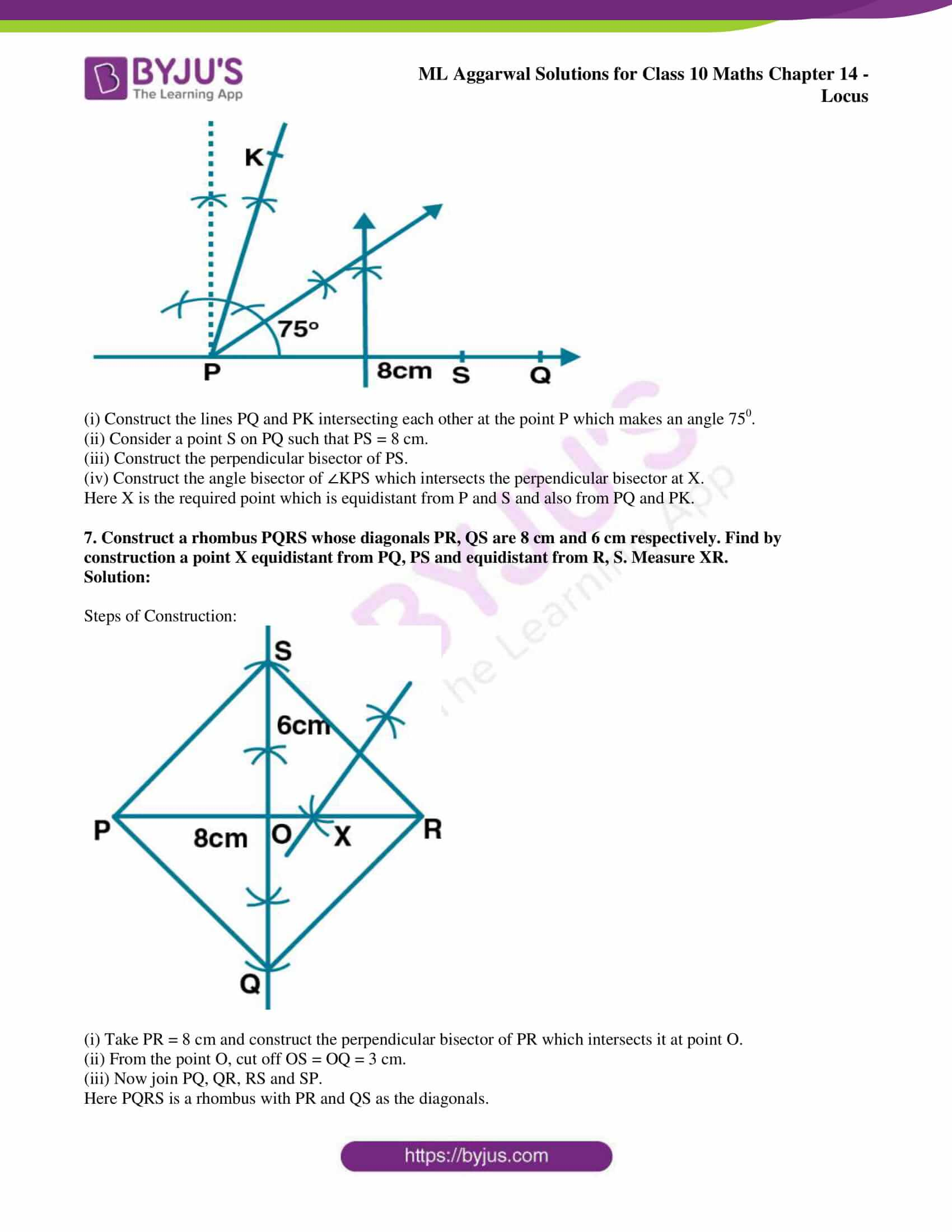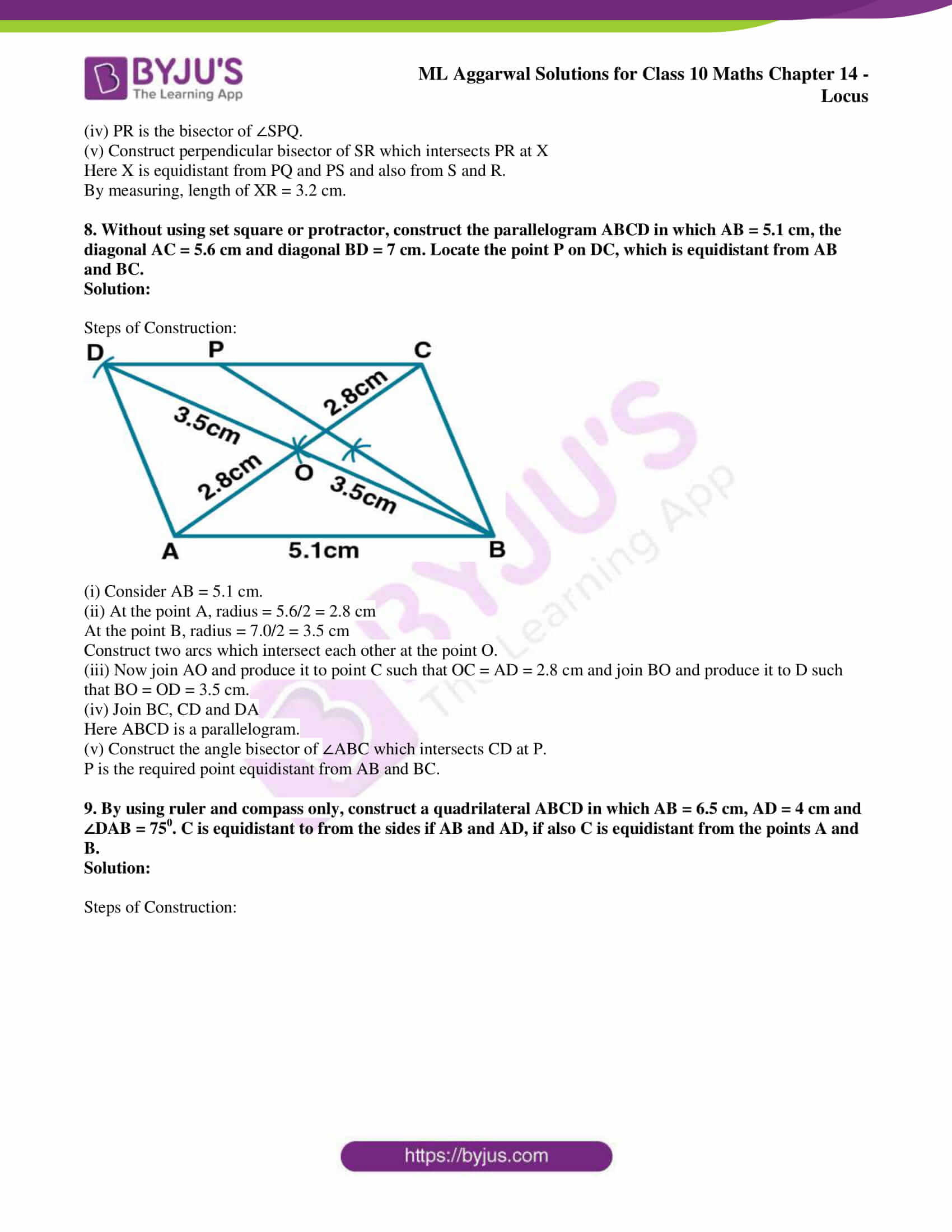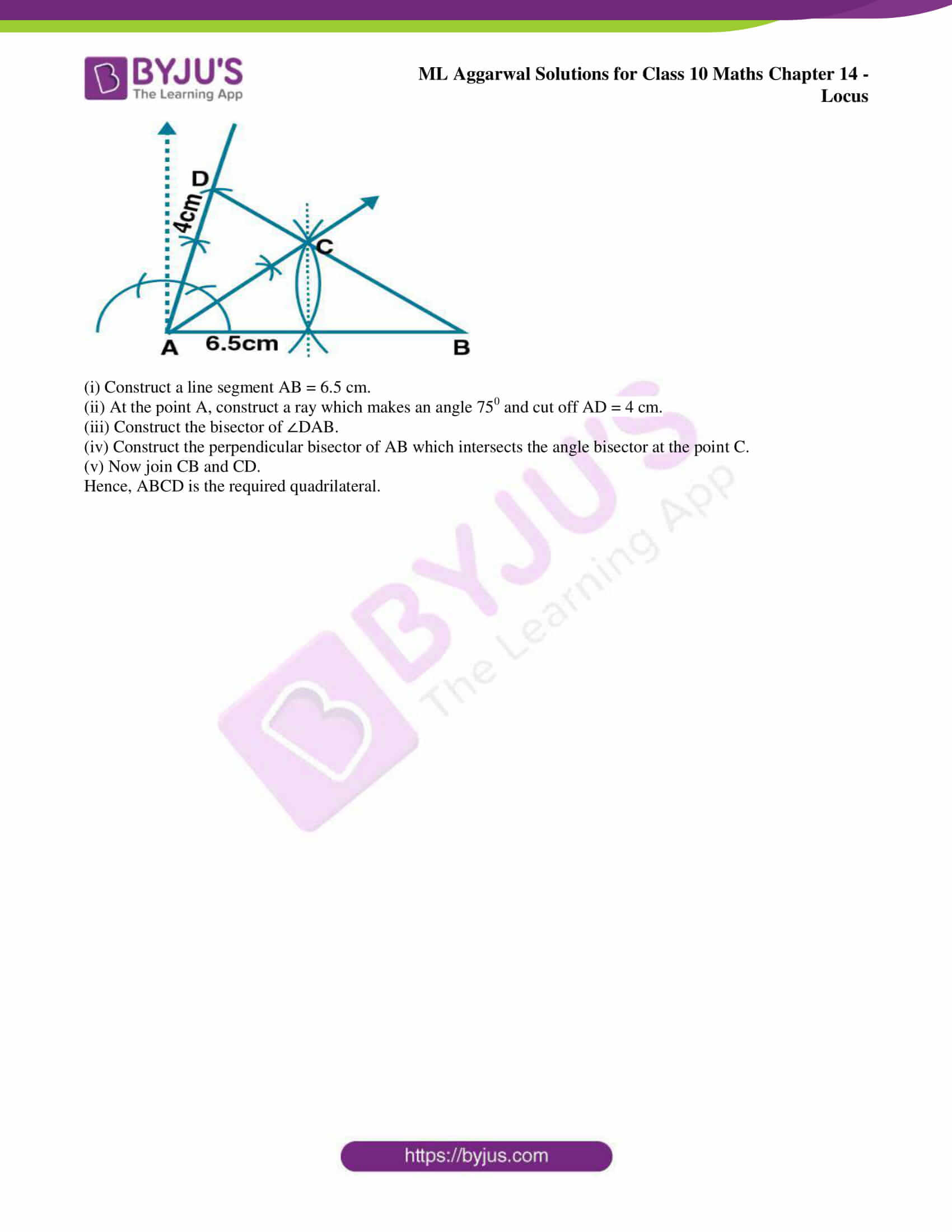## Access ML Aggarwal Solutions for Class 10 Maths Chapter 14: Locus

Exercise 14

1. A point moves such that its distance from a fixed line AB is always the same. What is the relation between AB and the path travelled by P?

Solution:

Consider point P which moves in such a way that it is at a fixed distance from the fixed line AB.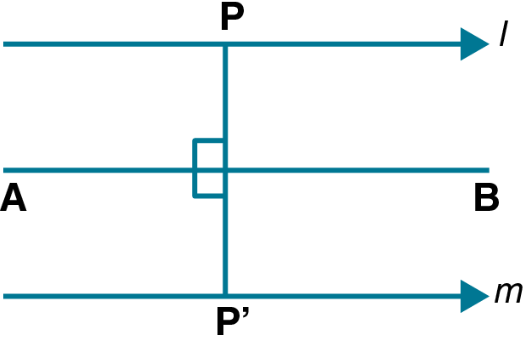Here it is a set of two lines l and m which is parallel to AB drawn on either side at an equal distance from it.

2. A point P moves so that its perpendicular distance from two given lines AB and CD are equal. State the locus of the point P.

Solution:

(i) We know that if two lines AB and CD are parallel, then the locus of point P which is equidistant from AB and CD is a line (l) in the midway of lines AB and CD and is parallel to them.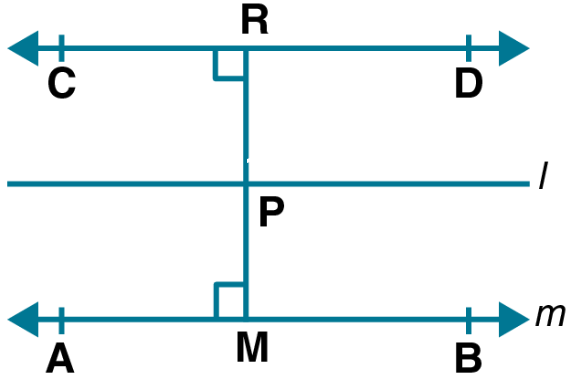(ii) If both AB and CD are intersecting lines, then the locus of point P will be l and m which is a pair of straight lines bisecting the angles between AB and CD.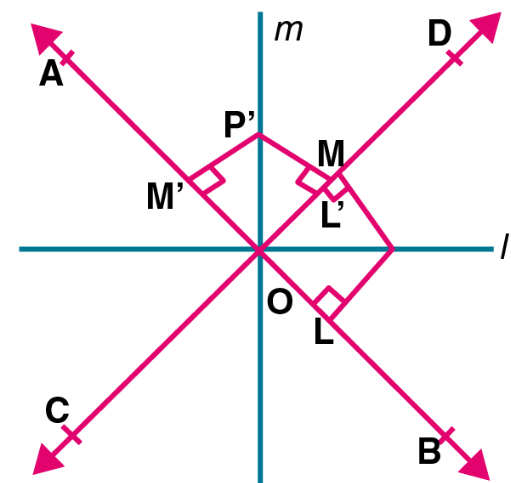3. P is a fixed point and a point Q moves such that the distance PQ is constant, what is the locus of the path traced out by the point Q?

Solution:

Consider P as a fixed point and Q as a moving point which is always at an equidistant from P.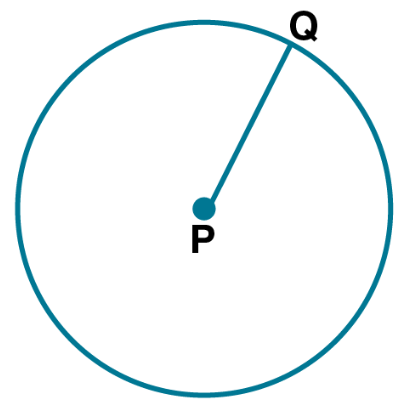Here P is the centre of the path of Q which is a circle.

We know that the distance between the points P and Q is the radius of the circle.

Therefore, locus of point Q is a circle with P as centre.

4. (i) AB is a fixed line. State the locus of the point P so that ∠APB = 900.

(ii) A, B are fixed points. State the locus of the point P so that ∠APB = 900.

Solution:

(i) It is given that

AB is a fixed line and P is a point such that ∠APB = 900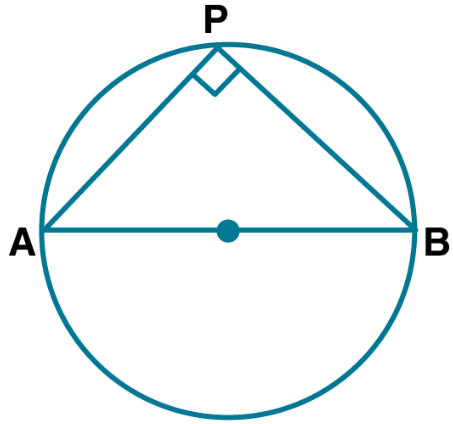Here the locus of point P will be the circle where AB is the diameter.

We know that the angle in a semi-circle is equal to 900 where ∠APB = 900

(ii) It is given that

AB is a fixed line and P is a point such that ∠APB = 600

Here the locus of point P will be a major segment of circle where AB is a chord.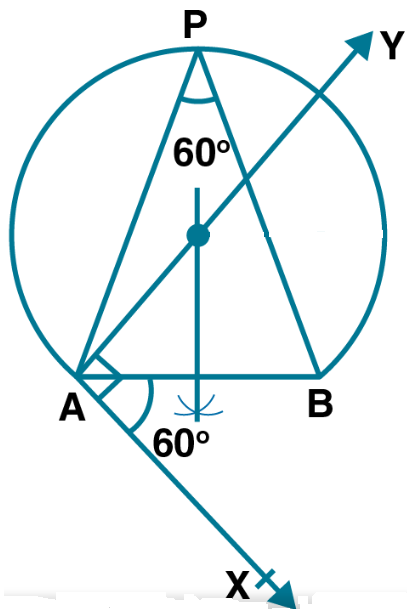5. Draw and describe the locus in each of the following cases:

(i) The locus of points at a distance 2.5 cm from a fixed line.

(ii) The locus of vertices of all isosceles triangles having a common base.

(iii) The locus of points inside a circle and equidistant from two fixed points on the circle.

(iv) The locus of centres of all circles passing through two fixed points.

(v) The locus of a point in rhombus ABCD which is equidistant from AB and AD.

(vi) The locus of a point in the rhombus ABCD which is equidistant from points A and C.

Solution:

(i) 1. Construct a line AB.

2. Construct lines l and m which are parallel to AB at a distance of 2.5 cm.

Here lines l and m are the locus of point P at a distance of 2.5 cm.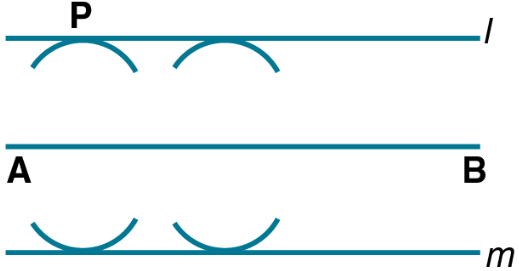(ii) It is given that

Δ ABC is an isosceles triangle where AB = AC.

Taking A as centre construct a perpendicular AD to BC.

Here AD is the locus of point A which are the vertices of Δ ABC

In Δ ABD and Δ ACD

It is given that

Hypotenuse AB = AC

According to RHS Axiom

Δ ABD = Δ ACD

BD = DC (c.p.c.t)

Therefore, locus of vertices of isosceles triangles having common base is the perpendicular bisector of BC.

(iii) 1. Construct a circle with O as centre.

2. Take points A and B on it and join them.

3. Construct a perpendicular bisector of AB which passes from point O and meets the circle at C.

Here CE which is the diameter is the locus of a point inside the circle and is equidistant from two points A and B at the circle.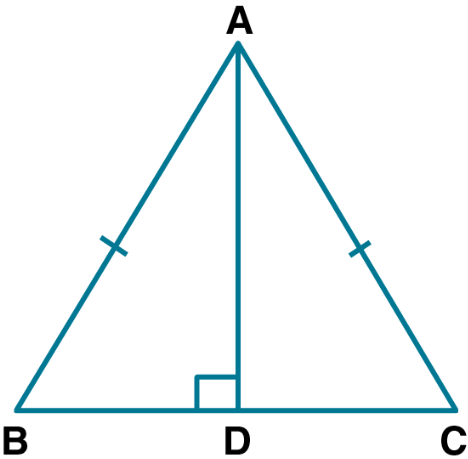(iv) Consider C1, C2 and C3 as the centres of circle which pass through A and B which are the two fixed points.

Construct a line XY which pass through the centres C1, C2 and C3.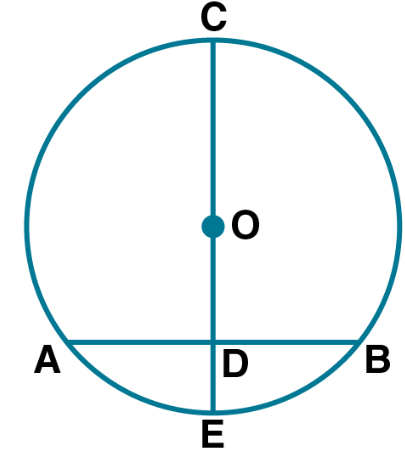Therefore, locus of centres of circles passing through two points A and B is the perpendicular bisector of the line segment which joins the two fixed points.

(v) In a rhombus ABCD, join AC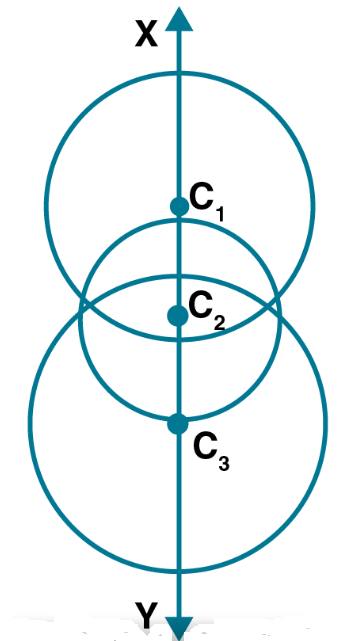Here AC is the diagonal of rhombus ABCD

We know that

AC bisects ∠A

Therefore, any point on AC is the locus which is equidistant from AB and AD.

(vi) In a rhombus ABCD, join BD.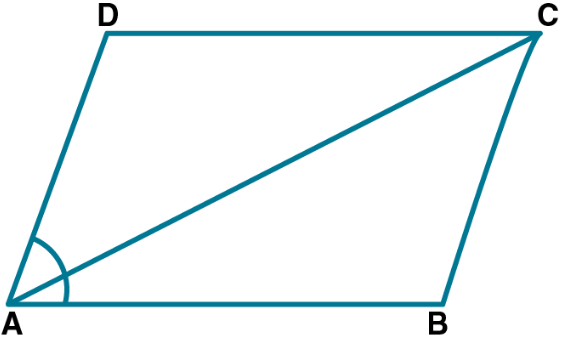Here BD is the locus of a point in the rhombus which is equidistant from A and C

We know that

Diagonal BD bisects ∠B and ∠D

So any point on BD will be equidistant from A and C.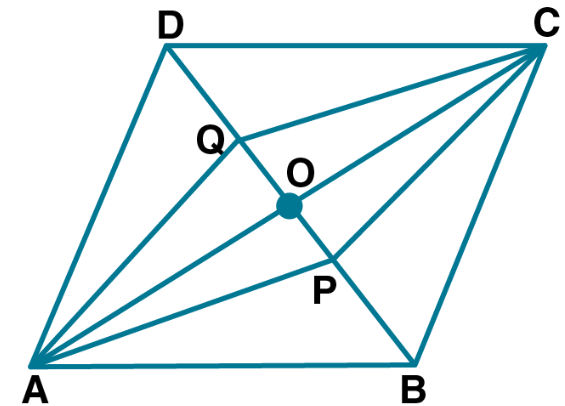6. Describe completely the locus of points in each of the following cases:

(i) mid-point of radii of a circle.

(ii) centre of a ball, rolling along a straight line on a level floor.

(iii) point in a plane equidistant from a given line.

(iv) point in a plane, at a constant distance of 5 cm from a fixed point (in the plane).

(v) centre of a circle of varying radius and touching two arms of ∠ADC.

(vi) centre of a circle of varying radius and touching a fixed circle, centre O, at a fixed point A on it.

(vii) centre of a circle of radius 2 cm and touching a fixed circle of radius 3 cm with centre O.

Solution:

(i) The locus of midpoints of the radii of a circle is another concentric circle with radius which is half of radius of given circle.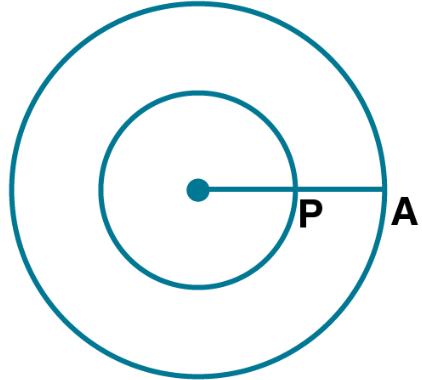(ii) Consider AB as a straight line on the ground and the ball is rolling on it

So the locus of the centre of the ball is a line which is parallel to the given line AB.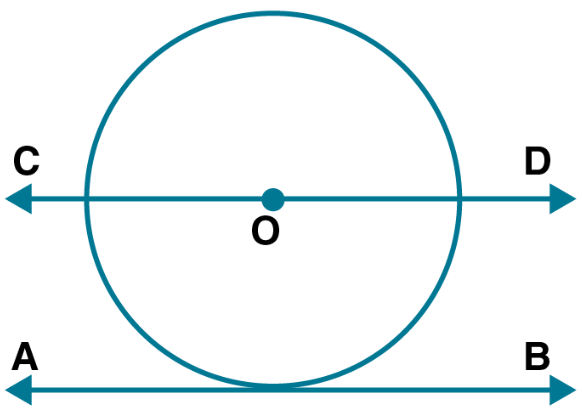(iii) We know that

AB is the given line and P is a point in the plane.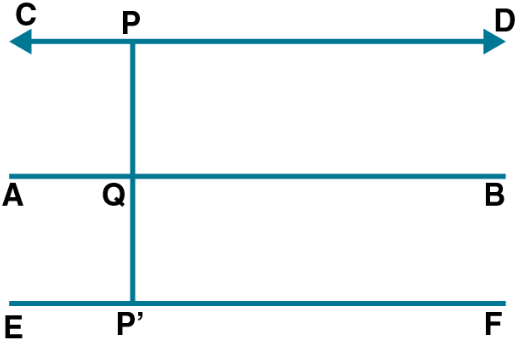From the point P, construct a line CD and another line EF from P’ parallel to AB.

Hence, CD and EF are the lines which are the locus of the point equidistant from AB.

(iv) Consider a point O and another point P where OP = 5 cm.

Taking O as centre and radius equal to OP, construct a circle.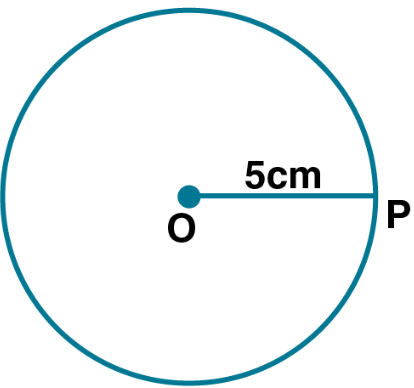Hence, this circle is the locus of point P which is at a distance of 5 cm from the given point O.

(v) Construct the bisector BX of ∠ABC.

So this bisector of an angle is the locus of the centre of a circle having different radii.

Here any point on BX is equidistant from BA and BC which are the arms of ∠ABC.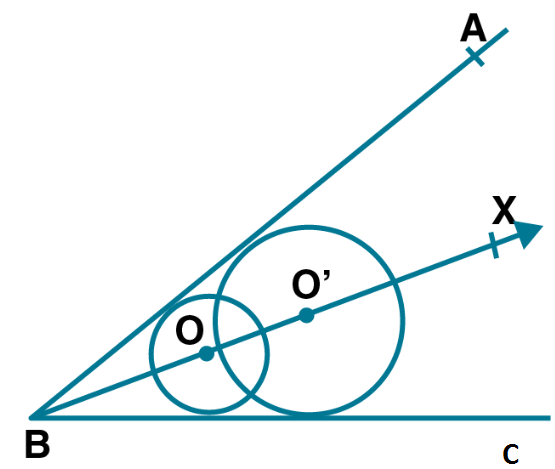(vi) Here a circle with O as centre is given with a point A on it.

The locus of the centre of a circle which touches the circle at fixed point A on it is the line joining the points O and A.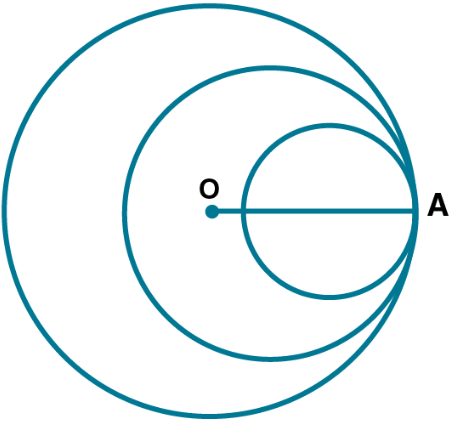(vii) (1) Here if the circle with 2 cm as radius touches the given circle externally then the locus of the centre of circle will be a concentric circle of radius 3 + 2 = 5 cm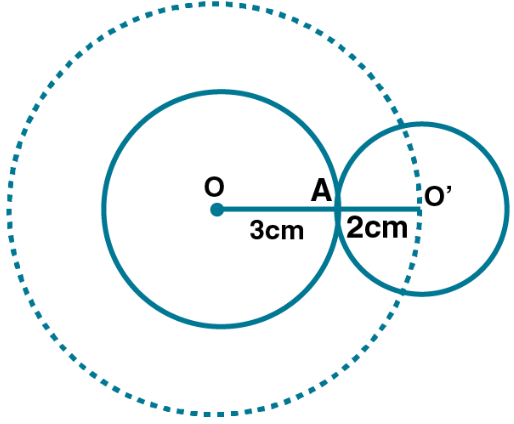(2) If the circle with 2 cm as radius touched the given circle with 3 cm as radius internally, then the locus of the centre of the circle will be a concentric circle of radius 3 – 2 = 1 cm.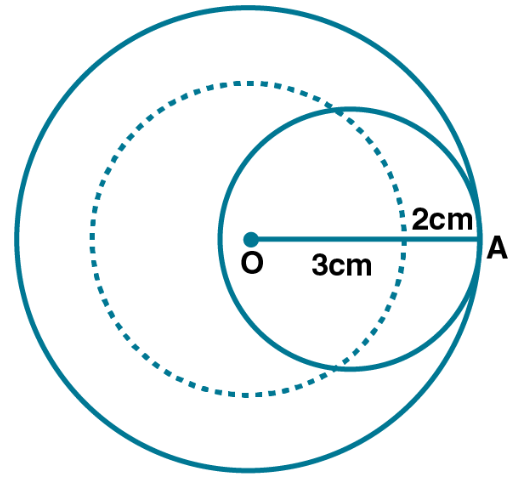7. Using ruler and compasses construct:

(i) a triangle ABC in which AB = 5.5 cm, BC = 3.4 cm and CA = 4.9 cm.

(ii) the locus of points equidistant from A and C.

Solution:

(i) Construct BC = 3.4 cm and mark the arcs 5.5 and 4.9 cm from the points B and C.

Now join A, B and C where ABC is the required triangle.

(ii) Construct a perpendicular bisector of AC.

(iii) Construct an angle of 900 at AB at point A which intersects the perpendicular bisector at point O.

Construct circle taking O as centre and OA as the radius.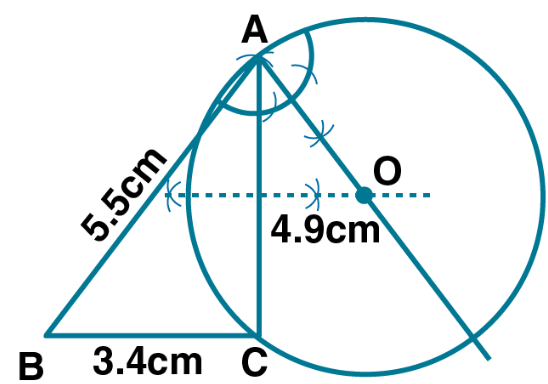8. Construct triangle ABC, with AB = 7 cm, BC = 8 cm and ∠ABC = 600. Locate by construction the point P such that:

(i) P is equidistant from B and C and

(ii) P is equidistant from AB and BC

(iii) Measure and record the length of PB.

Solution: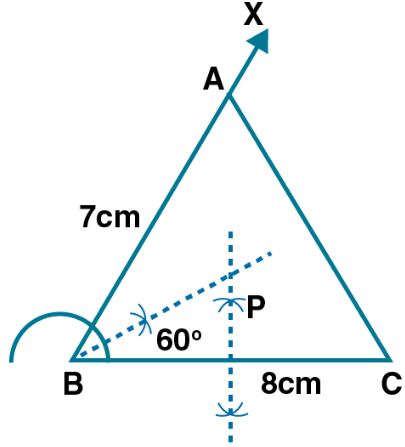(i) Consider BC = 8 cm as the long line segment.

At the point B construct a ray BX making an angle of 600 with BC

Now cut off BA = 7 cm and join AC.

Construct the perpendicular bisector of BC.

(ii) Construct the angle bisector of ∠B which intersect the perpendicular bisector of BC at P which is the required point.

(iii) By measuring, the length of PB = 4.6 cm.

9. A straight line AB is 8 cm long. Locate by construction the locus of a point which is:

(i) Equidistant from A and B.

(ii) Always 4 cm from the line AB.

(iii) Mark two points X and Y, which are 4 cm from AB and equidistant from A and B.

Name the figure AXBY.

Solution:

Steps of Construction: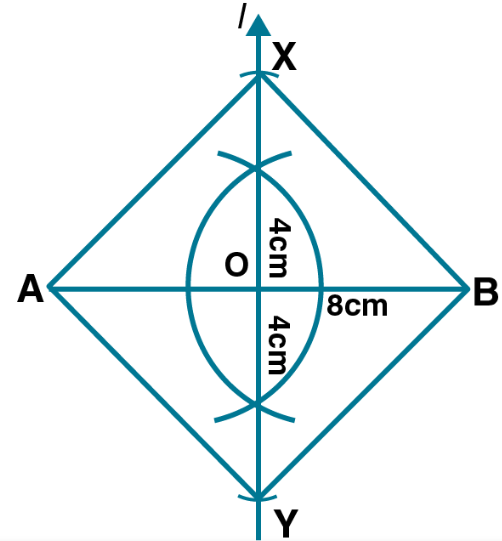(i) Construct a line segment AB = 8 cm.

(ii) Using compasses and ruler, construct a perpendicular bisector l of AB which intersects AB at the point O.

(iii) Here any point on l is equidistant from A and B.

(iv) Now cut off OX = OY = 4 cm. X and Y are the required loci which is equidistant from AB and also from point A and B.

(v) Join AX, XB, BY and YA.

The figures AXBY is square shaped as its diagonals are equal and bisect each other at right angles.

10. Use ruler and compasses only for this question.

(i) Construct Δ ABC, where AB = 3.5 cm, BC = 6 cm and ∠ABC = 600.

(ii) Construct the locus of points inside the triangle which are equidistant from BA and BC.

(iii) Construct the locus of points inside the triangle which are equidistant from B and C.

(iv) Mark the point P which is equidistant from AB, BC and also equidistant from B and C.

Measure and record the length of PB.

Solution:

We know that

In Δ ABC,

AB = 3.5 cm, BC = 6 cm and ∠ABC = 600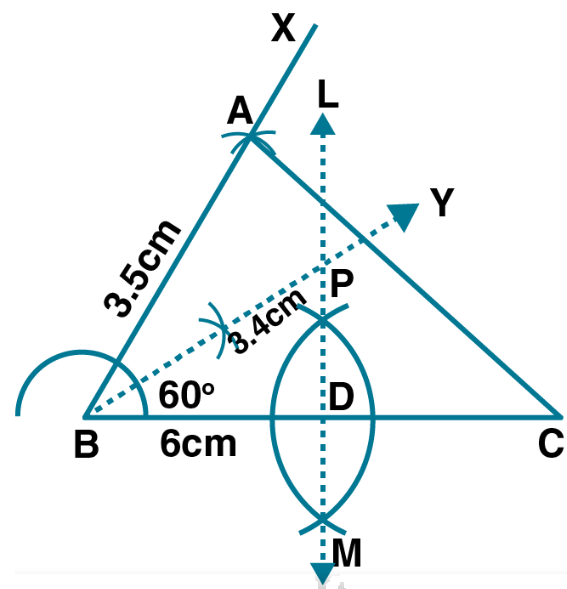Steps of Construction:

(i) Construct a line segment BC = 6 cm.

At the point B construct a ray BX which makes an angle 600 and cut off BA = 3.5 cm.

Now join AC.

Therefore, Δ ABC is the required triangle.

(ii) Construct the bisector BY of ∠ABC.

(iii) Construct a perpendicular bisector of BC which intersects BY at point P.

(iv) It is given that point P is equidistant from AB, BC and also equidistant from B and C.

By measuring, PB = 3.4 cm.

11. Construct a triangle ABC with AB = 5.5 cm, AC = 6 cm and ∠BAC = 1050. Hence:

(i) Construct the locus of points equidistant from BA and BC.

(ii) Construct the locus of points equidistant from B and C.

(iii) Mark the point which satisfies the above two loci as P. Measure and write the length of PC.

Solution:

Steps of Construction: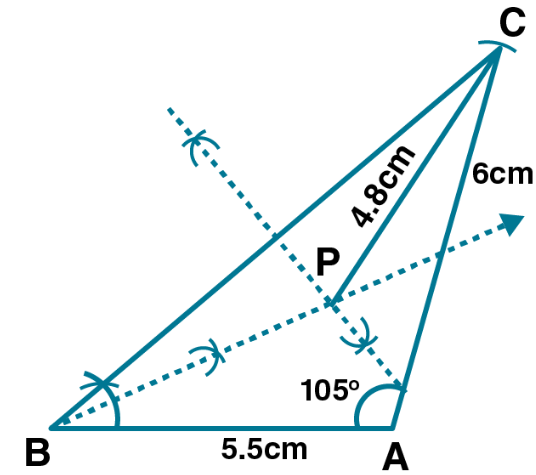Construct a triangle ABC with AB = 5.5 cm, AC = 6 cm and ∠BAC = 1050.

(i) The points which are equidistant from BA and BC lies on the bisector of ∠ABC.

(ii) The points equidistant from B and C lies on the perpendicular bisector of BC.

Construct perpendicular bisector of BC.

P is the point of intersection of the bisector of ∠ABC and the perpendicular bisector of BC.

(iii) By measuring, the required length of PC = 4.8 cm.

12. In the diagram, A, B and C are fixed collinear points; D is a fixed point outside the line. Locate: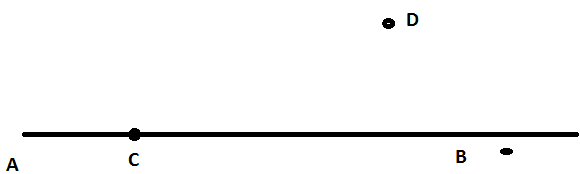(i) the point P on AB such that CP = DP.

(ii) the points Q such that CQ = DQ = 3 cm. How many such points are possible?

(iii) the points R on AB such that DR = 4 cm. How many such points are possible?

(iv) the points S such that CS = DS and S is 4 cm away from the line CD. How many such points are possible?

(v) Are the points P, Q, R collinear?

(vi) Are the points P, Q, S collinear?

Solution:

Here the points A, B and C are collinear and D is any point which is outside AB.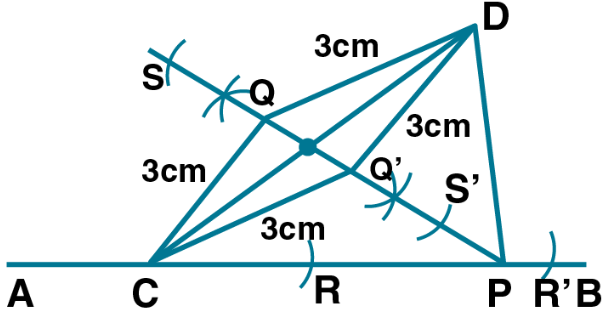(i) Join CD.

Construct the perpendicular bisector of CD which meets AB in P.

Here P is the required point such that CP = DP.

(ii) Taking C and D as centres, construct two arcs with radius 3 cm which intersect each other at Q and Q’

Therefore, there are two points Q and Q’ which are equidistant from C and D.

(iii) Taking D as centre and 4 cm radius construct an arc which intersects AB at R and R’

Here R and R’ are the two points on AB.

(iv) Taking C and D as centre construct arcs with a 4 cm radius which intersect each other in S and S’.

Hence, there can be two such points equidistant from C and D.

(v) No, the points P, Q, R are not collinear.

(vi) Yes, the points P, Q, S are collinear.

13. Points A, B and C represent position of three towers such that AB = 60 mm, BC = 73 mm and CA = 52 mm. Taking a scale of 10 m to 1 cm, make an accurate drawing of Δ ABC. Find by drawing, the location of a point which is equidistant from A, B and C and its actual distance from any of the towers.

Solution:

It is given that

AB = 60 mm = 6 cm

BC = 73 mm = 7.3 cm

CA = 52 mm = 5.2 cm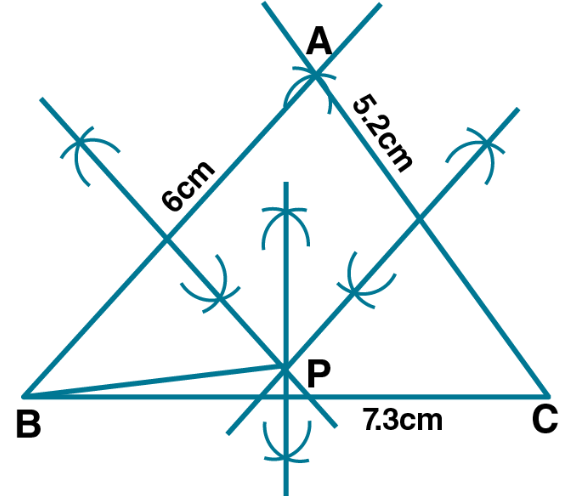(i) Construct a line segment BC = 7.3 cm.

(ii) Taking B as centre and 6 cm radius and C as centre and 5.2 cm radius, construct two arcs which intersect each other at the point A.

(iii) Now join AB and AC.

(iv) Construct perpendicular bisector of AB, BC and CA which intersect each other at the point P and join PB.

Here P is equidistant from A, B and C on measuring PB = 3.7 cm.

The actual distance is 37 m.

14. Draw two intersecting lines to include an angle of 300. Use ruler and compasses to locate points which are equidistant from these lines and also 2 cm away from their point of intersection. How many such points exist?

Solution:

(i) AB and CD are the two lines which intersect each other at the point O.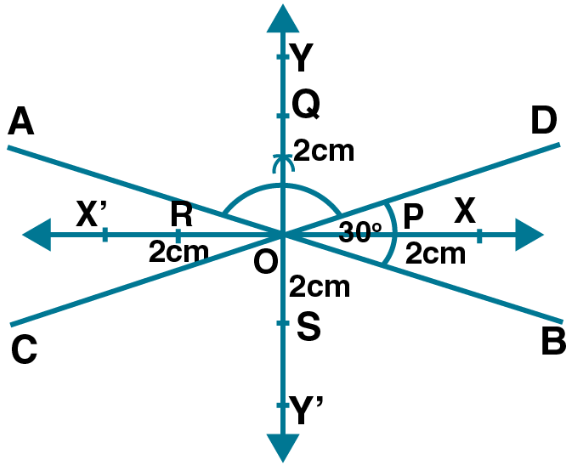(ii) Construct the bisector of ∠BOD and ∠AOD.

(iii) Taking O as centre and 2 cm radius mark points on the bisector of angles at P, Q, R and S respectively.

Therefore, there are four points equidistant from AB and CD and 2 cm from O which is the point of intersection of AB and CD.

15. Without using set square or protractor, construct the quadrilateral ABCD in which ∠BAD = 450, AD = AB = 6 cm, BC = 3.6 cm and CD = 5 cm.

(i) Measure ∠BCD.

(ii) Locate the point P on BD which is equidistant from BC and CD.

Solution:

(i) Consider AB = 6 cm long.

(ii) At point A, construct the angle of 450 and cut off AD = 6 cm.

(iii) Taking D as centre and 5 cm radius and B as centre and 3.5 cm radius construct two arcs which intersect each other at point C.

(iv) Now join CD, CB and BD.

Here ABCD is the required quadrilateral.

(v) By measuring ∠BCD = 650.

(vi) Construct the bisector of ∠BCD which intersects BD at the point P.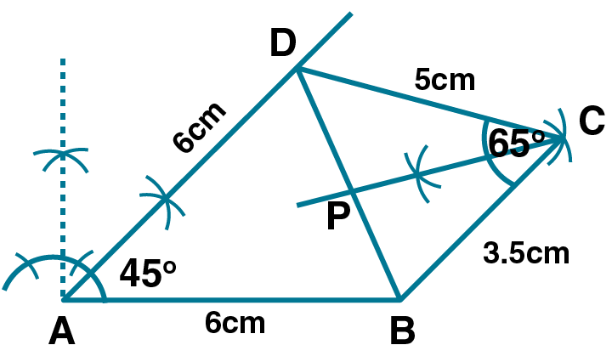Hence, P is the required point equidistant from BD and CD.

16. Without using set square or protractor, construct rhombus ABCD with sides of length 4 cm and diagonal AC of length 5 cm. Measure ∠ABC. Find the point R on AD such that RB = RC. Measure the length of AR.

Solution: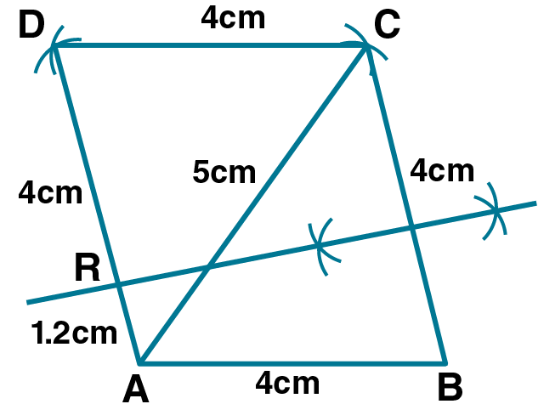(i) Construct AB = 4 cm.

(ii) Taking A as centre, construct an arc of radius 5 cm and with B as centre construct another arc of 4 cm radius which intersect each other at the point C.

(iii) Now join AC and BD.

(iv) With A and C as centre, construct two arcs of 4 cm radius which intersect each other on D.

Hence, ABCD is the required rhombus and by measure ∠ABC = 780.

(vi) Construct perpendicular bisector of BC which intersects AD at the point R.

By measuring the length of AR = 1.2 cm.

17. Without using set-squares or protractor construct:

(i) Triangle ABC, in which AB = 5.5 cm, BC = 3.2 cm and CA = 4.8 cm.

(ii) Draw the locus of a point which moves so that it is always 2.5 cm from B.

(iii) Draw the locus of a point which moves so that it is equidistant from the sides BC and CA.

(iv) Mark the point of intersection of the loci with the letter P and measure PC.

Solution:

Steps of Construction: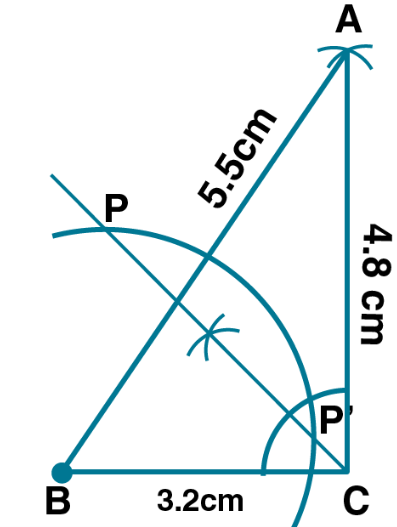(i) Construct BC = 3.2 cm long.

(ii) Taking B as centre and 5.5 cm radius and C as centre and 4.8 cm radius construct arcs intersecting each other at the point A.

(iii) Now join AB and AC.

(iv) Construct the bisector of ∠BCA.

(v) Taking B as centre and 2.5 cm radius, construct an arc which intersects the angle bisector of ∠BCA at P and P’.

Here P and P’ are the two loci which satisfy the given condition.

By measuring CP and CP’

CP = 3.6 cm and CP’ = 1.1 cm.

18. By using ruler and compasses only, construct an isosceles triangle ABC in which BC = 5 cm, AB = AC and ∠BAC = 900. Locate the point P such that:

(i) P is equidistant from the sides BC and AC.

(ii) P is equidistant from the points B and C.

Solution:

Steps of Construction: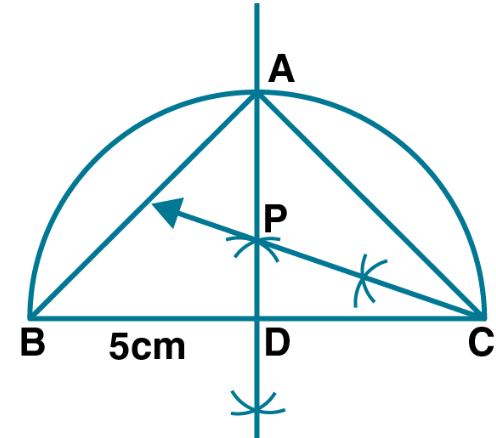(i) Construct BC = 5 cm and bisect it at point D.

(ii) Taking BC as diameter, construct a semicircle.

(iii) At the point D, construct a perpendicular intersecting the circle at the point A.

(iv) Now join AB and AC.

(v) Construct the angle bisector of C which intersects the perpendicular at the point P

Here P is the required point.

19. Using ruler and compasses only, construct a quadrilateral ABCD in which AB = 6 cm, BC = 5 cm, ∠B = 600, AD = 5 cm and D is equidistant from AB and BC. Measure CD.

Solution:

Steps of Construction: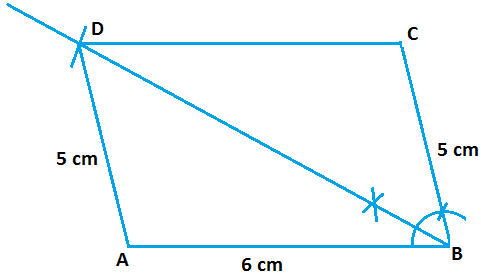(i) Construct AB = 6 cm.

(ii) At point B, construct angle 600 and cut off BC = 5 cm.

(iii) Construct the angle bisector of ∠B.

(iv) Taking A as centre and 5 cm radius construct an arc which intersects the angle bisector of ∠B at D.

(v) Now join AD and DC.

Here ABCD is the required quadrilateral.

By measuring CD = 5.3 cm.

20. Construct an isosceles triangle ABC such that AB = 6 cm, BC = AC = 4 cm. Bisect ∠C internally and mark a point P on this bisector such that CP = 5 cm. Find the points Q and R which are 5 cm from P and also 5 cm from the line AB.

Solution:

Steps of Construction: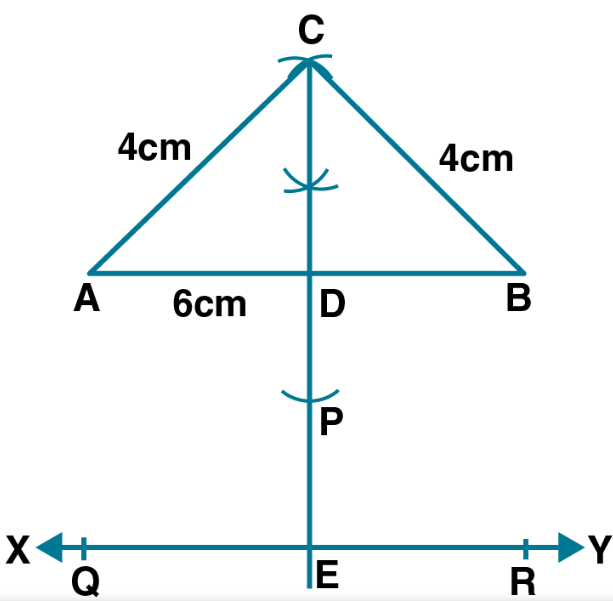(i) Construct a line AB = 6 cm.

(ii) Taking A and B as centre and 4 cm radius, construct two arcs which intersect each other at the point C.

(iii) Now join CA and CB.

(iv) Construct the bisector of ∠C and cut off CP = 5 cm.

(v) Construct a line XY parallel to AB at 5 cm distance.

(vi) At point P, construct arcs of radius 5 cm each which intersects the line XY at Q and R.

Therefore, Q and R are the required points.

21. Use ruler and compasses only for this question. Draw a circle of radius 4 cm and mark two chords AB and AC of the circle of length 6 cm and 5 cm respectively.

(i) Construct the locus of points, inside the circle, that are equidistant from A and C. Prove your construction.

(ii) Construct the locus of points, inside the circle, that are equidistant from AB and AC.

Solution:

Steps of Construction: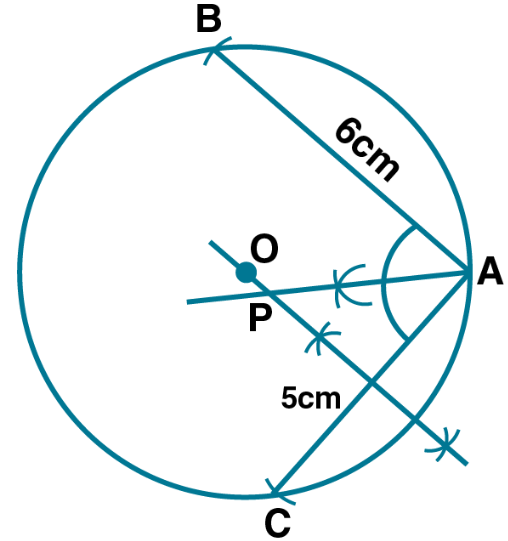(i) Taking O as centre and 4 cm radius construct a circle.

(ii) Mark a point A on this circle.

(iii) Taking A and centre and 6 cm radius construct an arc which cuts the circle at B.

(iv) Again with 5 cm radius, construct another arc which cuts the circle at C.

(vi) Construct the perpendicular bisector of AC.

Here any point on it will be equidistant from A and C.

(vii) Construct the angle bisector of ∠A which intersects the perpendicular bisector of AC at the point P.

Hence, P is the required locus.

22. Ruler and compasses only may be used in this question. All construction lines and arcs must be clearly shown and be of sufficient length and clarity to permit assessment.

(i) Construct a triangle ABC, in which BC = 6 cm, AB = 9 cm and ∠ABC = 600.

(ii) Construct the locus of all points, inside Δ ABC, which are equidistant from B and C.

(iii) Construct the locus of the vertices of the triangle with BC as base, which are equal in area to Δ ABC.

(iv) Mark the point Q, in your construction, which would make Δ QBC equal in area to Δ ABC and isosceles.

(v) Measure and record the length of CQ.

Solution:

Steps of Construction: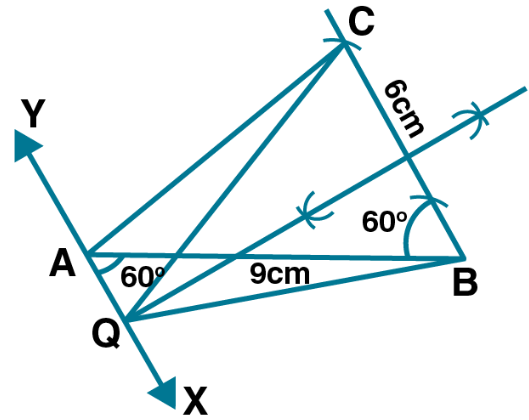(i) Construct AB = 9 cm.

(ii) At the point B construct an angle of 600 and cut off BC = 6 cm.

(iii) Now join AC.

(iv) Construct perpendicular bisector of BC.

Here all the points on it will be equidistant from B and C.

(v) From the point A, construct a line XY which is parallel to BC.

(vi) Produce the perpendicular bisector of BC to meet the line XY at the point Q.

(vii) Now join QC and QB.

The area of Δ QBC is equal to the area of Δ ABC as these are on the same base and between the same parallel lines.

By measuring, length of CQ = 8.2 cm.

Chapter-Test

1. Draw a straight line AB of length 8 cm. Draw the locus of all points which are equidistant from A and B. Prove your statement.

Solution:

(i) Construct a line segment AB = 8 cm.

(ii) Construct the perpendicular bisector of AB which intersects AB at the point D.

Here every point P on it will be equidistant from A and B.

(iii) Take a point P on the perpendicular bisector.

(iv) Now join PA and PB.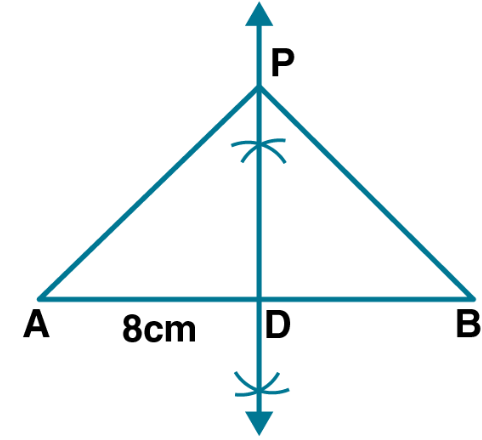Proof:

In Δ PAD and Δ PBD

PD = PD is common

As D is the midpoint of AB

We know that

∠PDA = ∠PDB = 900

Δ PAD ≅ Δ PBD as per SAS axiom of congruency

PA = PB (c. p. c. t)

In the same way, we can prove that any other point which lies on the perpendicular bisector of AB is equidistant from A and B.

Therefore, it is proved.

2. A point P is allowed to travel in space. State the locus of P so that it always remains at a constant distance from a fixed point C.

Solution:

It is given that

A point P is allowed to travel in space and is at a constant distance from a fixed point C.

Therefore, its locus is a sphere.

3. Draw a line segment AB of length 7 cm. Construct the locus of a point P such that area of triangle PAB is 14 cm2.

Solution:

It is given that

Length of AB = 7 cm (base)

Area of triangle PAB = 14 cm2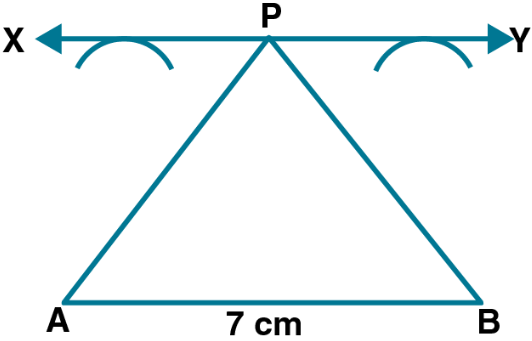We know that

Height = (area × 2)/ base

Substituting the values

= (14 × 2)/ 7

= 4 cm

Construct a line XY which is parallel to AB and at a distance of 4 cm.

Take any point P on XY

Now join PA and PB

Area of triangle PAB = 14 cm2

Therefore, locus of P is the line XY which is parallel to AB at a distance of 4 cm.

4. Draw a line segment AB of length 12 cm. Mark M, the mid-point of AB. Draw and describe the locus of a point which is

(i) at a distance of 3 cm from AB.

(ii) at a distance of 5 cm from the point M. Mark the points P, Q, R, S which satisfy both the above conditions. What kind of quadrilateral is PQRS? Compute the area of the quadrilateral PQRS.

Solution:

Steps of Construction: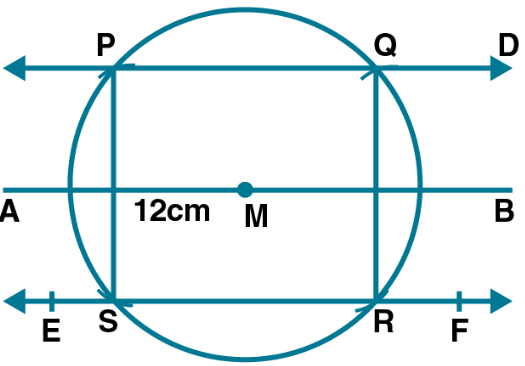(i) Construct a line AB = 12 cm.

(ii) Take M as the midpoint of line AB.

(iii) Construct straight lines CD and EF which is parallel to AB at 3 cm distance.

(iv) Taking M as centre and 5 cm radius construct areas which intersect CD at P and Q and EF at R and S.

(v) Now join QR and PS.

Here PQRS is a rectangle where the length PQ = 8 cm

So the area of rectangle PQRS = PQ × RS

We get

= 8 × 6

= 48 cm2

5. AB and CD are two intersecting lines. Find the position of a point which is at a distance of 2 cm from AB and 1.6 cm from CD.

Solution:

(i) AB and CD are two intersecting lines which intersect each other at the point O.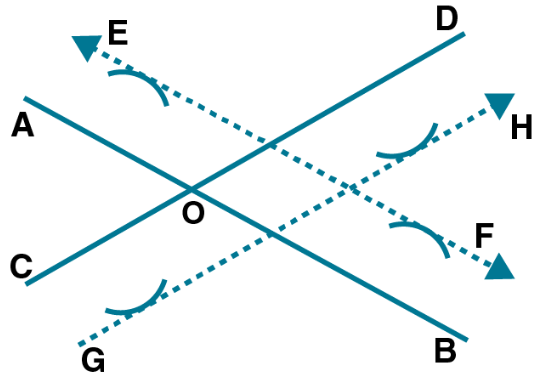(ii) Construct a line EF which is parallel to AB and GH which is parallel to CD intersecting each other at the point P.

Hence, P is the required point.

6. Two straight lines PQ and PK cross each other at P at an angle of 750. S is a stone on the road PQ, 800 m from P towards Q. By drawing a figure to scale 1 cm = 100 m, locate the position of a flagstaff X, which is equidistant from P and S, and is also equidistant from the road.

Solution:

We know that

1 cm = 100 cm

800 m = 8 cm

Steps of Construction: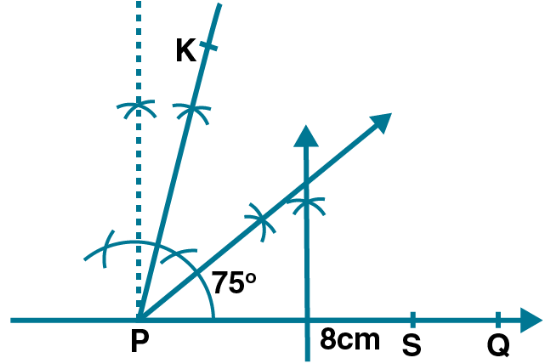(i) Construct the lines PQ and PK intersecting each other at the point P which makes an angle 750.

(ii) Consider a point S on PQ such that PS = 8 cm.

(iii) Construct the perpendicular bisector of PS.

(iv) Construct the angle bisector of ∠KPS which intersects the perpendicular bisector at X.

Here X is the required point which is equidistant from P and S and also from PQ and PK.

7. Construct a rhombus PQRS whose diagonals PR, QS are 8 cm and 6 cm respectively. Find by construction a point X equidistant from PQ, PS and equidistant from R, S. Measure XR.

Solution:

Steps of Construction: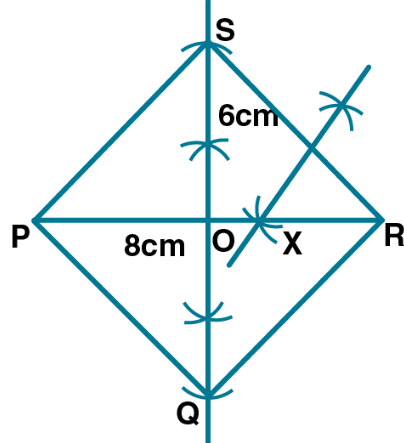(i) Take PR = 8 cm and construct the perpendicular bisector of PR which intersects it at point O.

(ii) From the point O, cut off OS = OQ = 3 cm.

(iii) Now join PQ, QR, RS and SP.

Here PQRS is a rhombus with PR and QS as the diagonals.

(iv) PR is the bisector of ∠SPQ.

(v) Construct perpendicular bisector of SR which intersects PR at X

Here X is equidistant from PQ and PS and also from S and R.

By measuring, length of XR = 3.2 cm.

8. Without using set square or protractor, construct the parallelogram ABCD in which AB = 5.1 cm, the diagonal AC = 5.6 cm and diagonal BD = 7 cm. Locate the point P on DC, which is equidistant from AB and BC.

Solution:

Steps of Construction: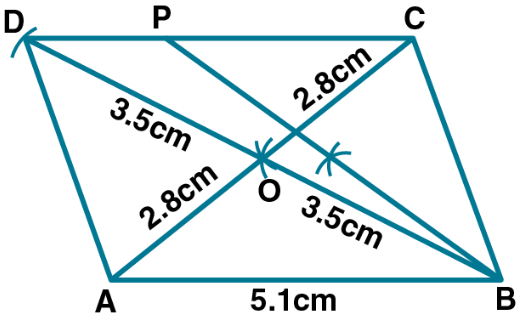(i) Consider AB = 5.1 cm.

(ii) At the point A, radius = 5.6/2 = 2.8 cm

At the point B, radius = 7.0/2 = 3.5 cm

Construct two arcs which intersect each other at the point O.

(iii) Now join AO and produce it to point C such that OC = AD = 2.8 cm and join BO and produce it to D such that BO = OD = 3.5 cm.

(iv) Join BC, CD and DA

Here ABCD is a parallelogram.

(v) Construct the angle bisector of ∠ABC which intersects CD at P.

P is the required point equidistant from AB and BC.

9. By using ruler and compass only, construct a quadrilateral ABCD in which AB = 6.5 cm, AD = 4 cm and ∠DAB = 750. C is equidistant to from the sides if AB and AD, if also C is equidistant from the points A and B.

Solution:

Steps of Construction: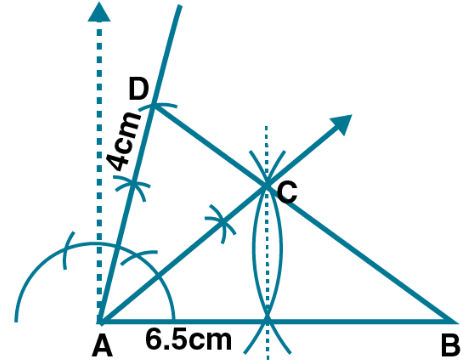(i) Construct a line segment AB = 6.5 cm.

(ii) At the point A, construct a ray which makes an angle 750 and cut off AD = 4 cm.

(iii) Construct the bisector of ∠DAB.

(iv) Construct the perpendicular bisector of AB which intersects the angle bisector at the point C.

(v) Now join CB and CD.

Hence, ABCD is the required quadrilateral.